# Lakhmir Singh Solutions Class 10 Physics Chapter 5 Refraction of Light

Refraction is defined as the bending of the wave when it enters a medium where its speed is different. Refraction of light is one such phenomenon. According to laws of refraction of light, the incident ray, the refracted ray and the normal are all in the same plane.

Also, the ratio between the sine of the angle of incidence and the sine of the angle of refraction is equal to a constant. Twinkling of stars at night and the formation of mirages are the effects of refraction, while the formation of a rainbow is an example of refraction of light. Also, other concepts such as refraction of light through spherical lenses (concave and convex lenses) and through a prism help in understanding the behaviour of light.

## Download PDF Of Lakhmir Singh Solutions for Class 10 Physics Chapter 5 Refraction of Light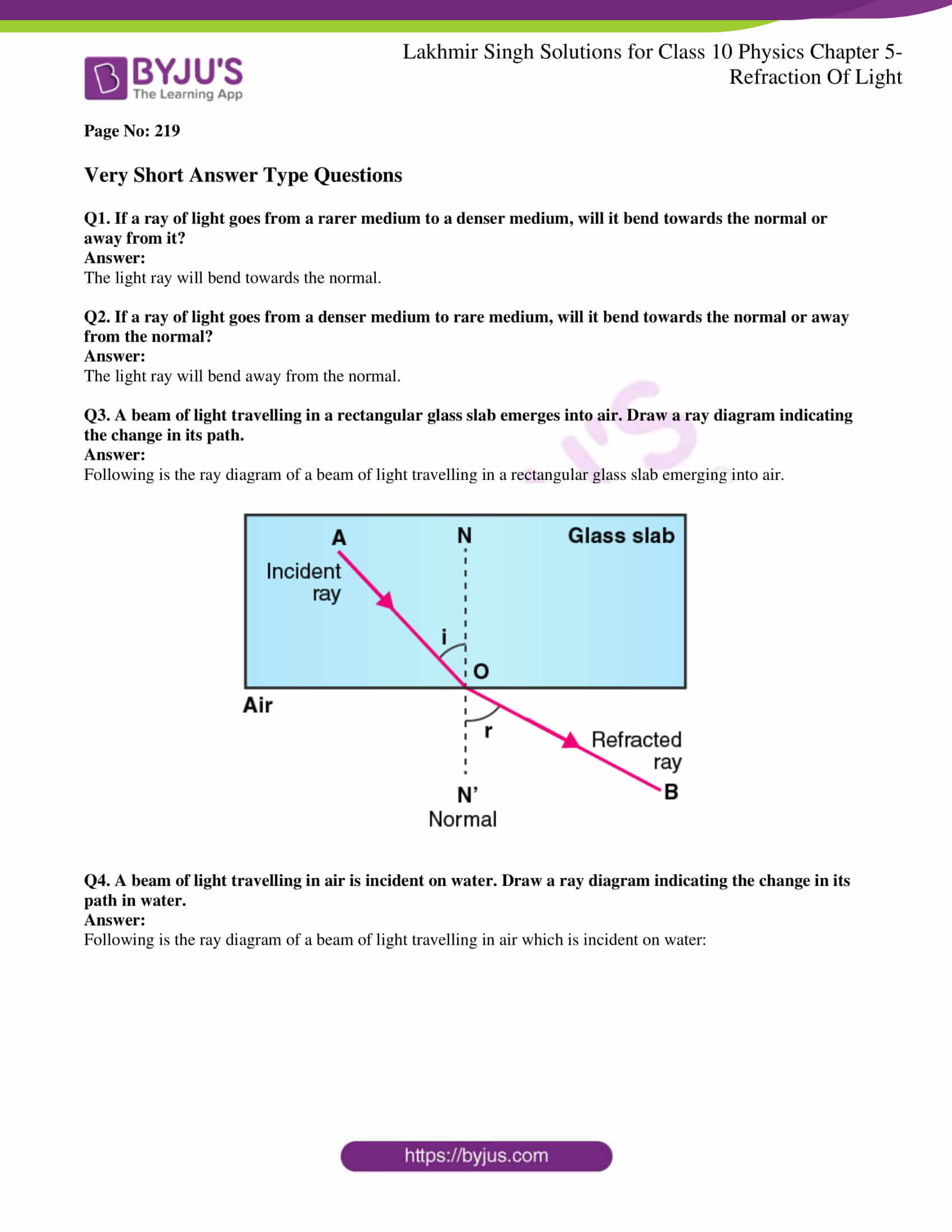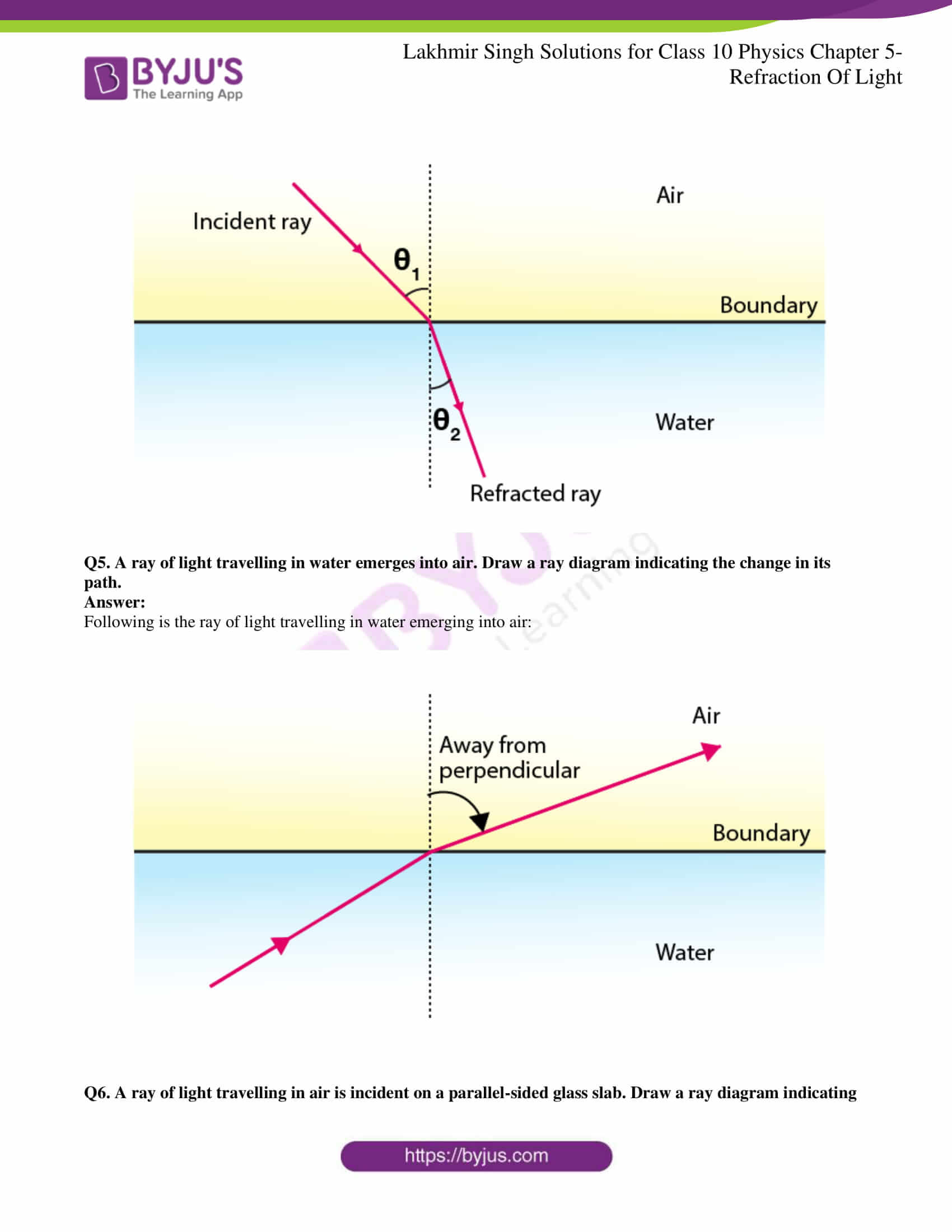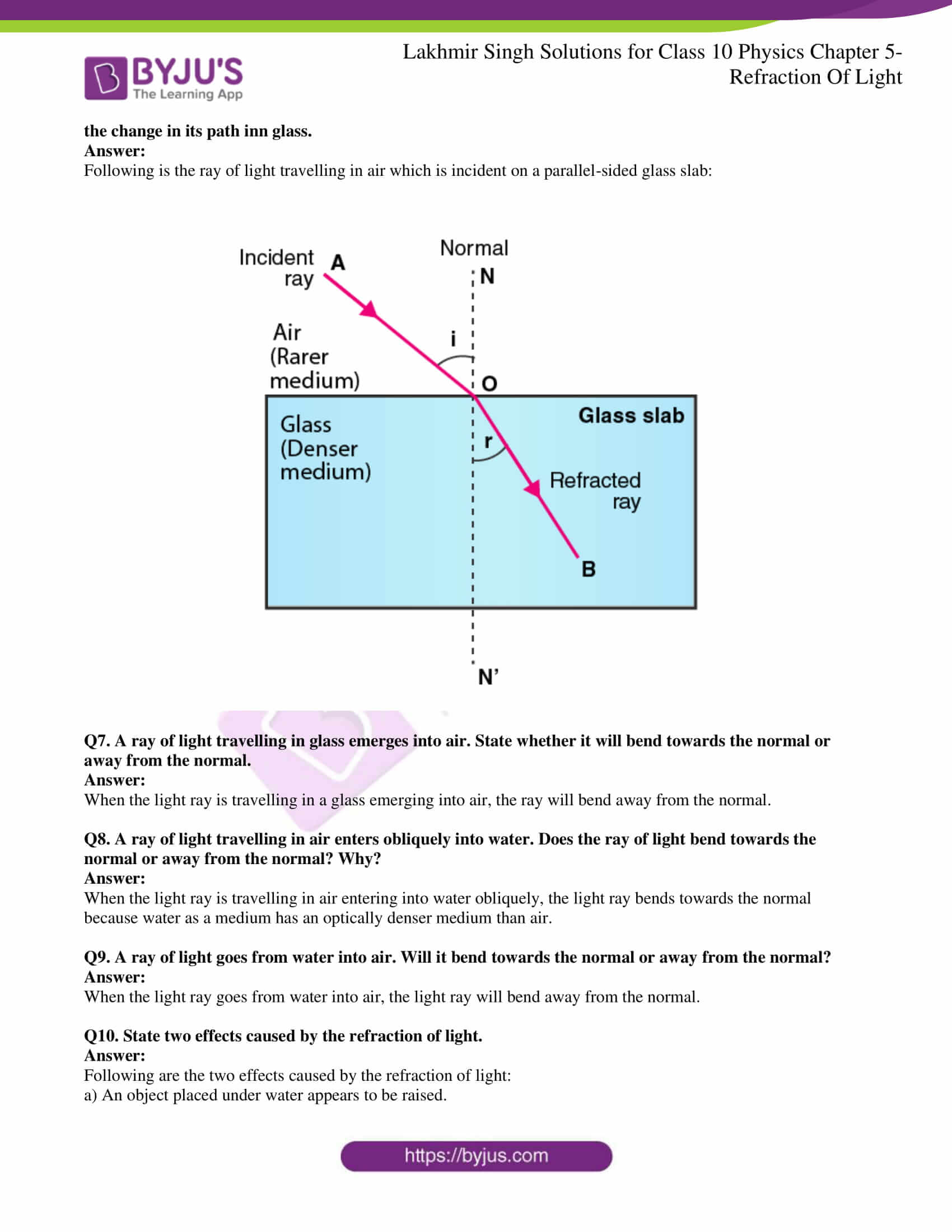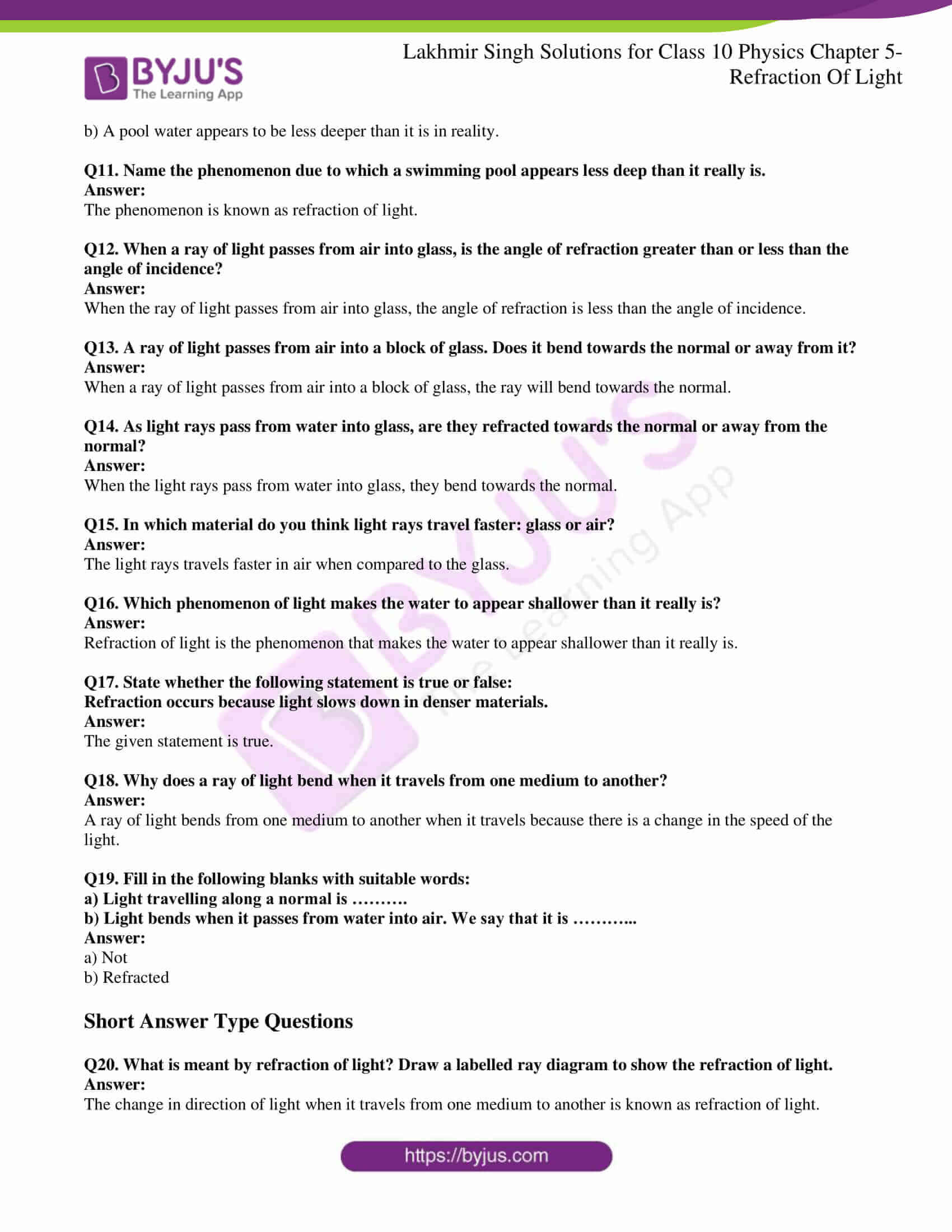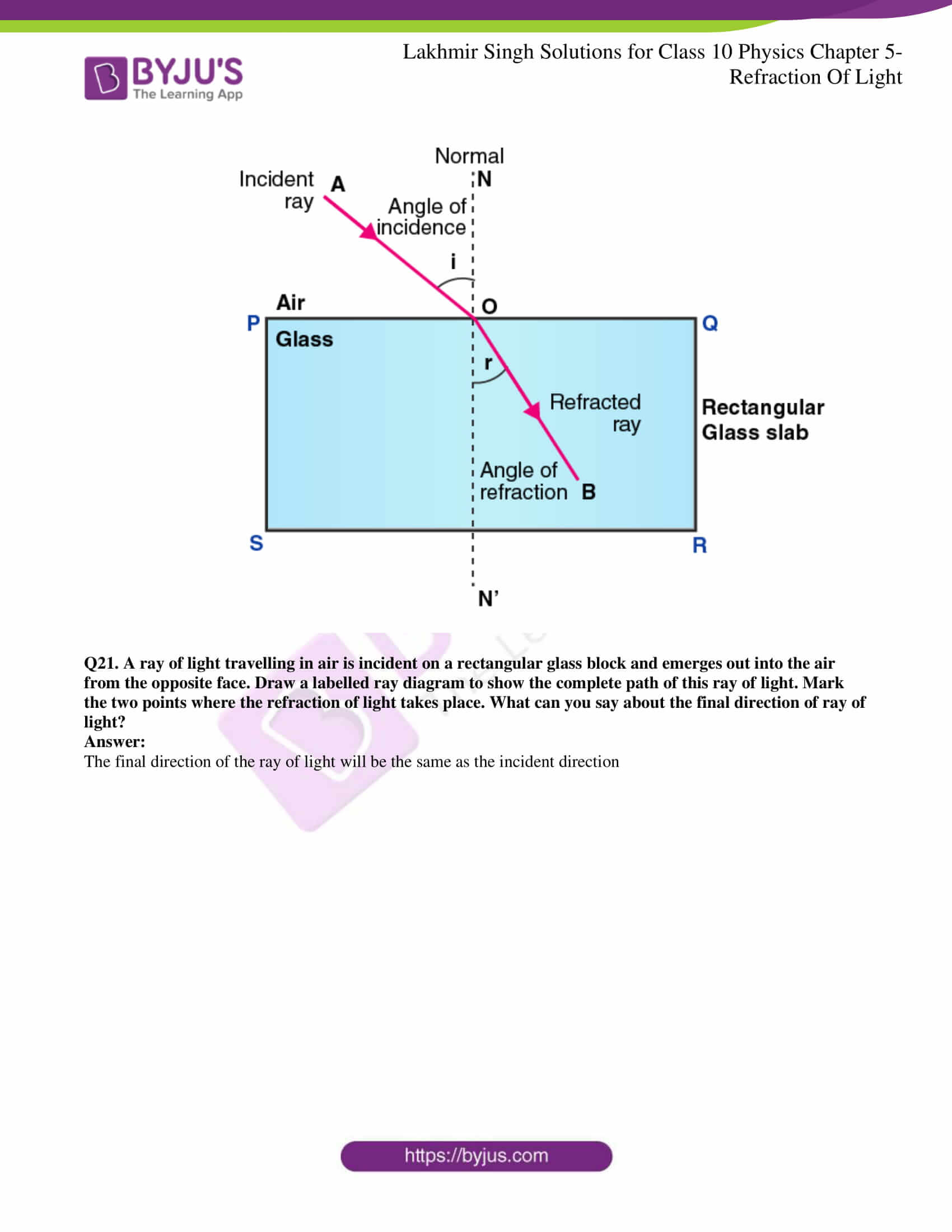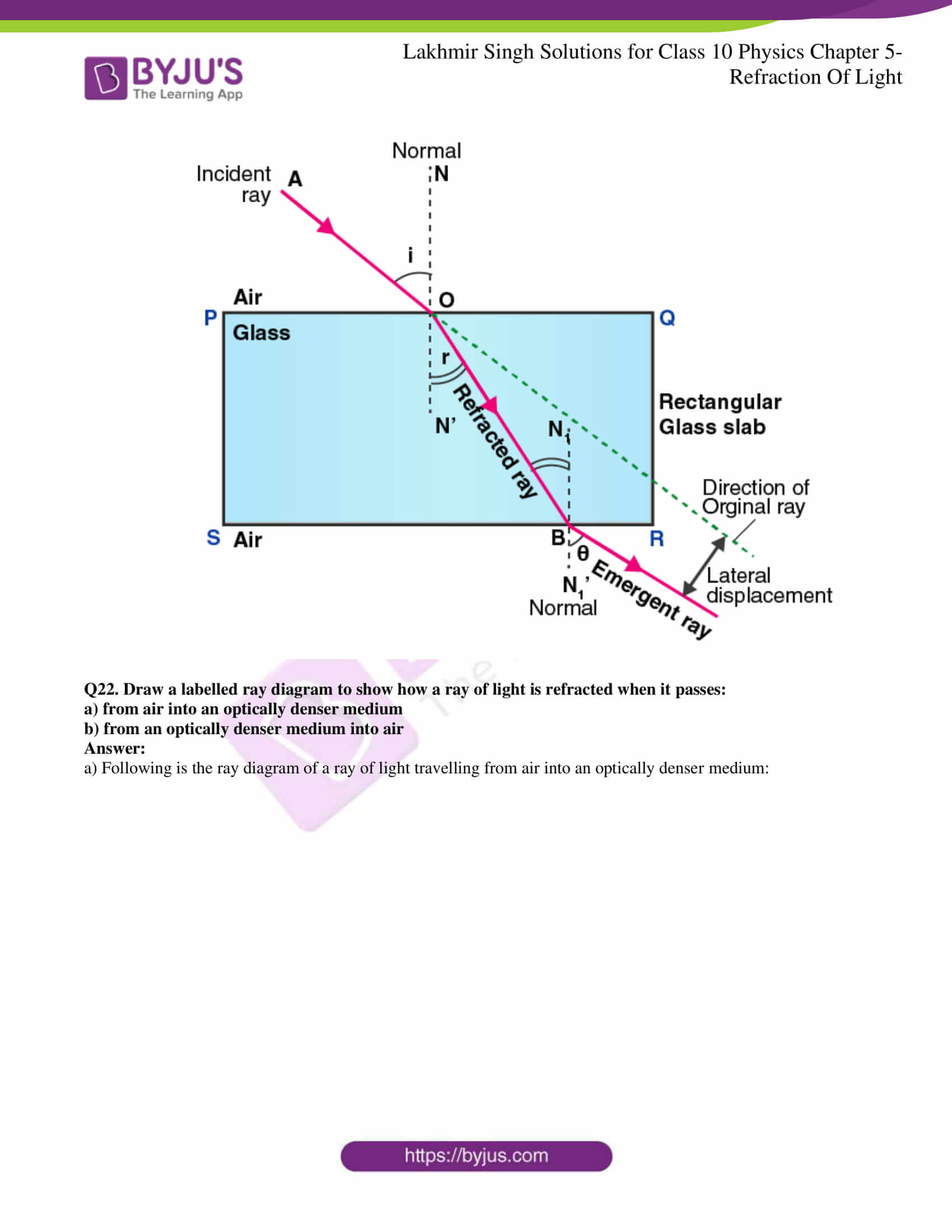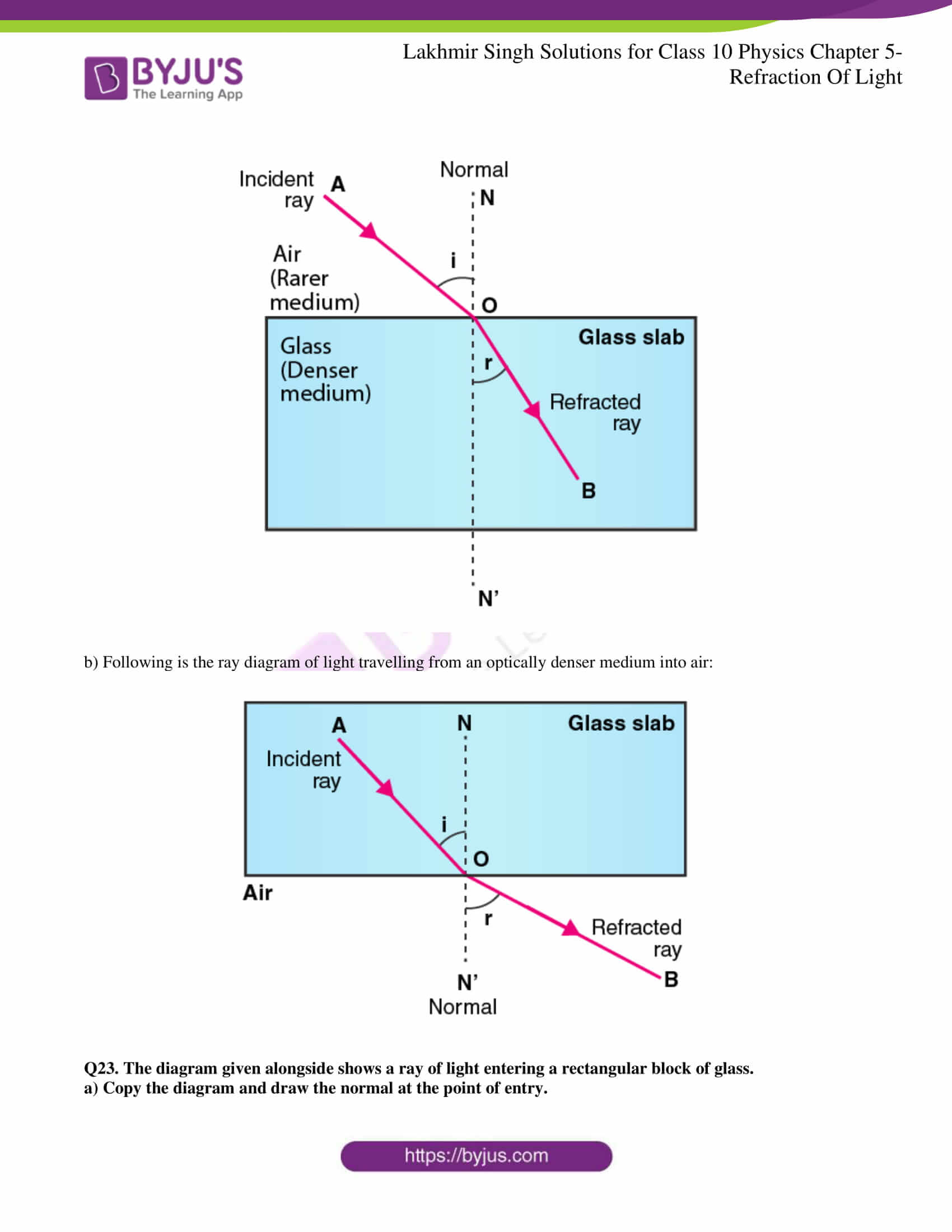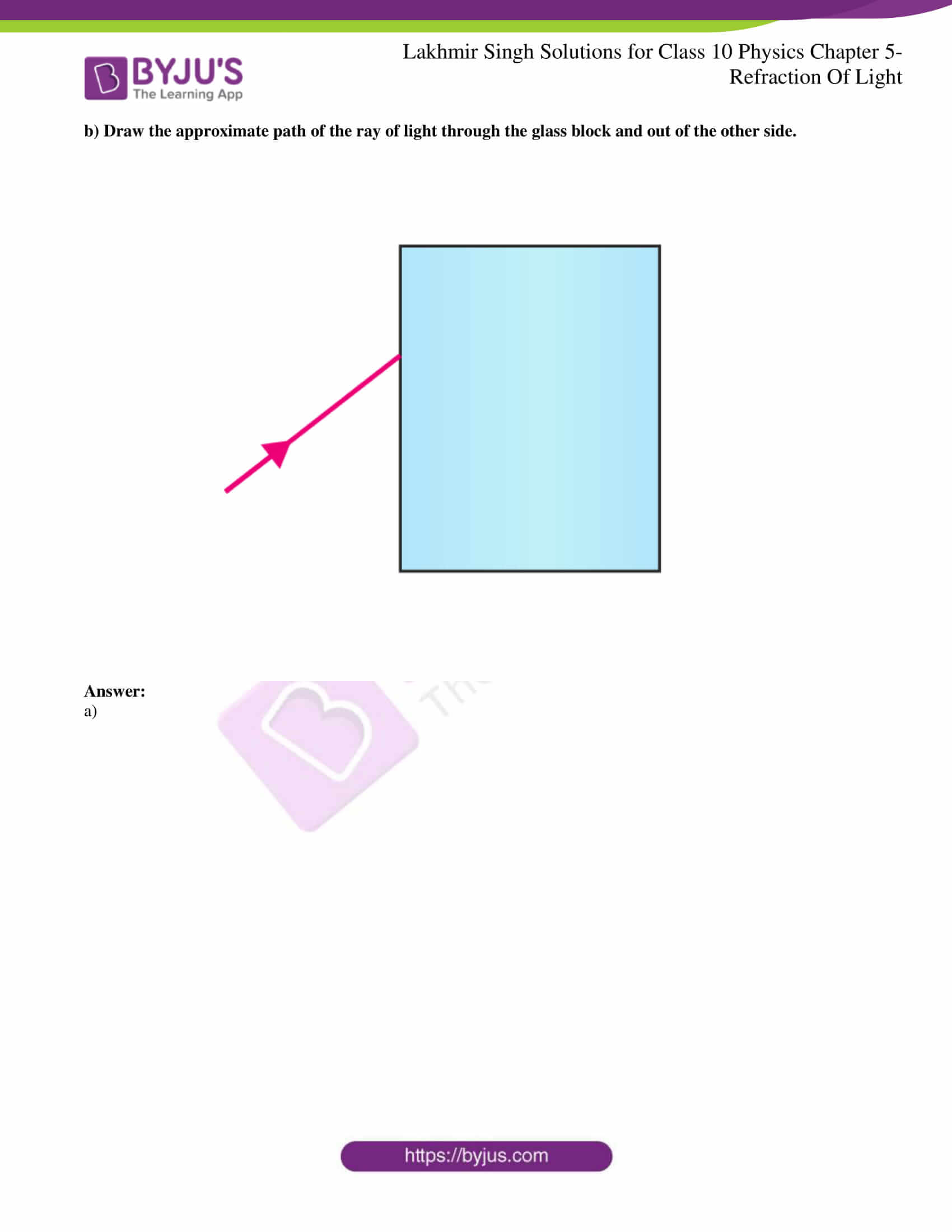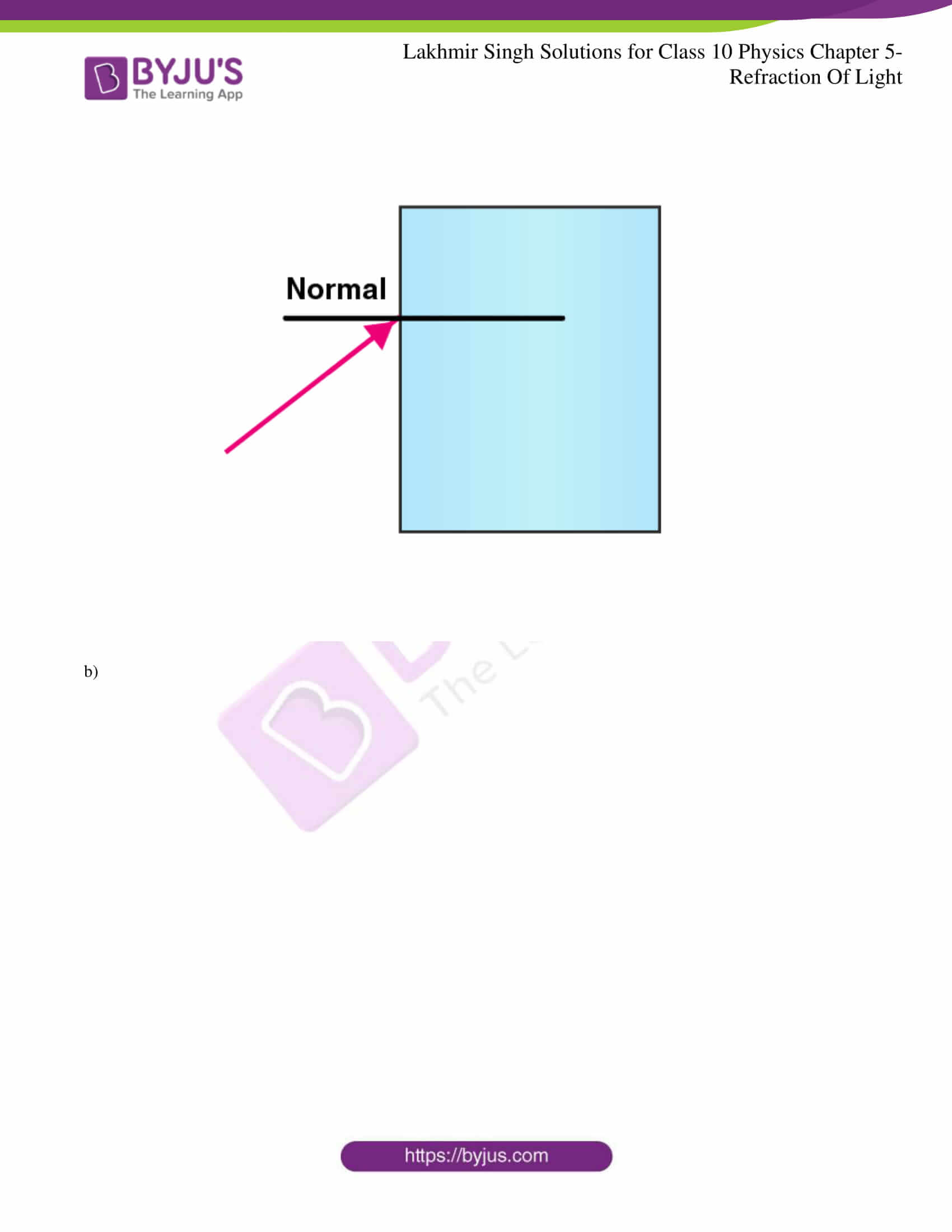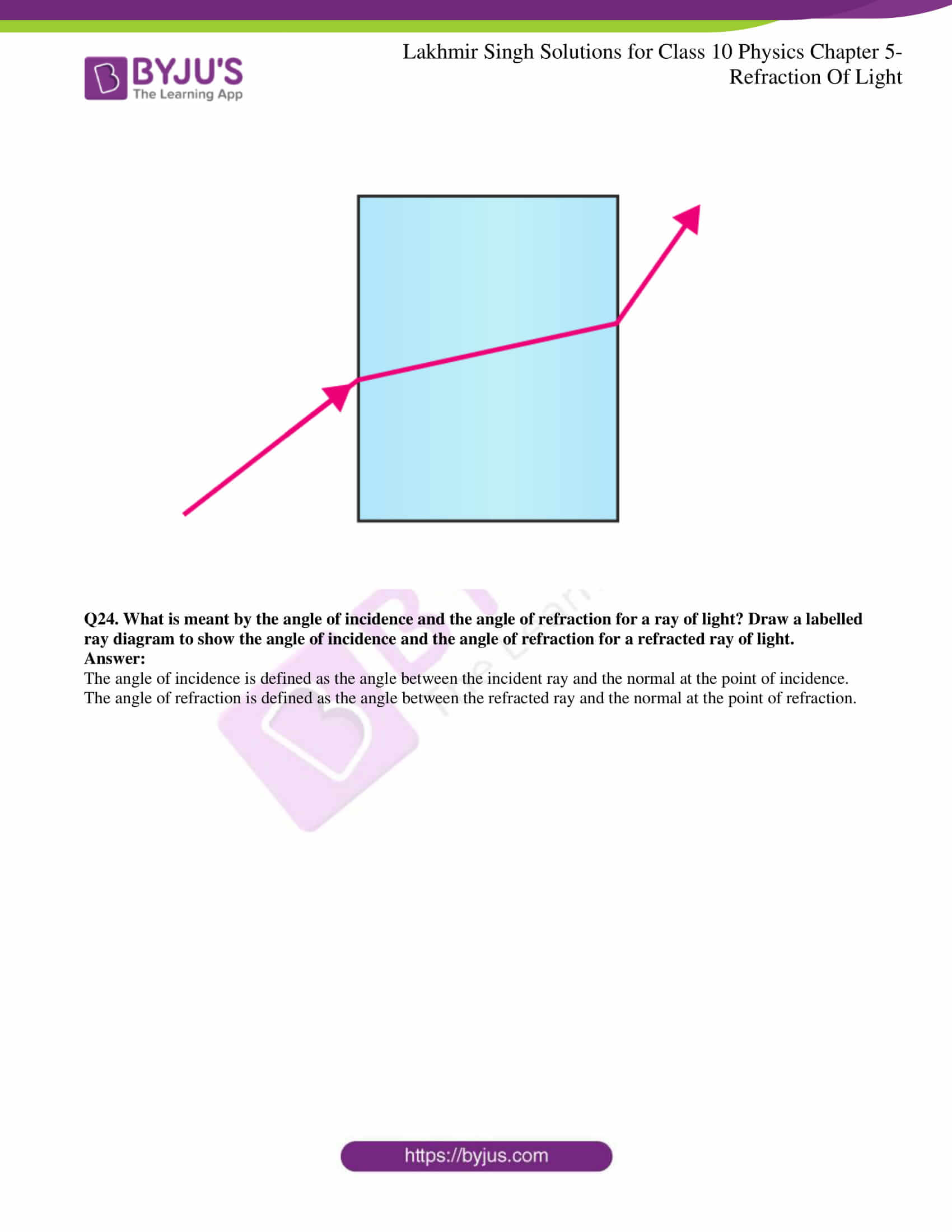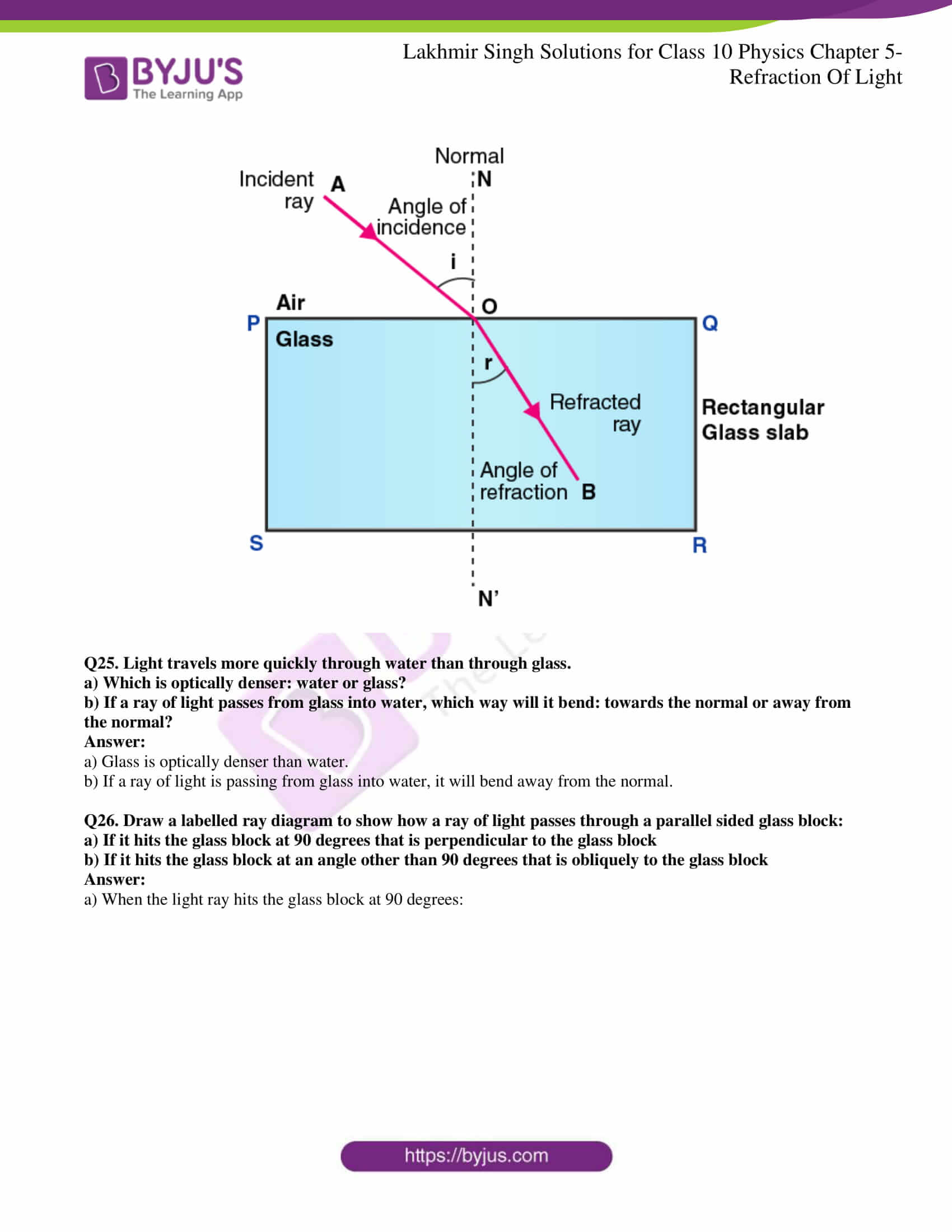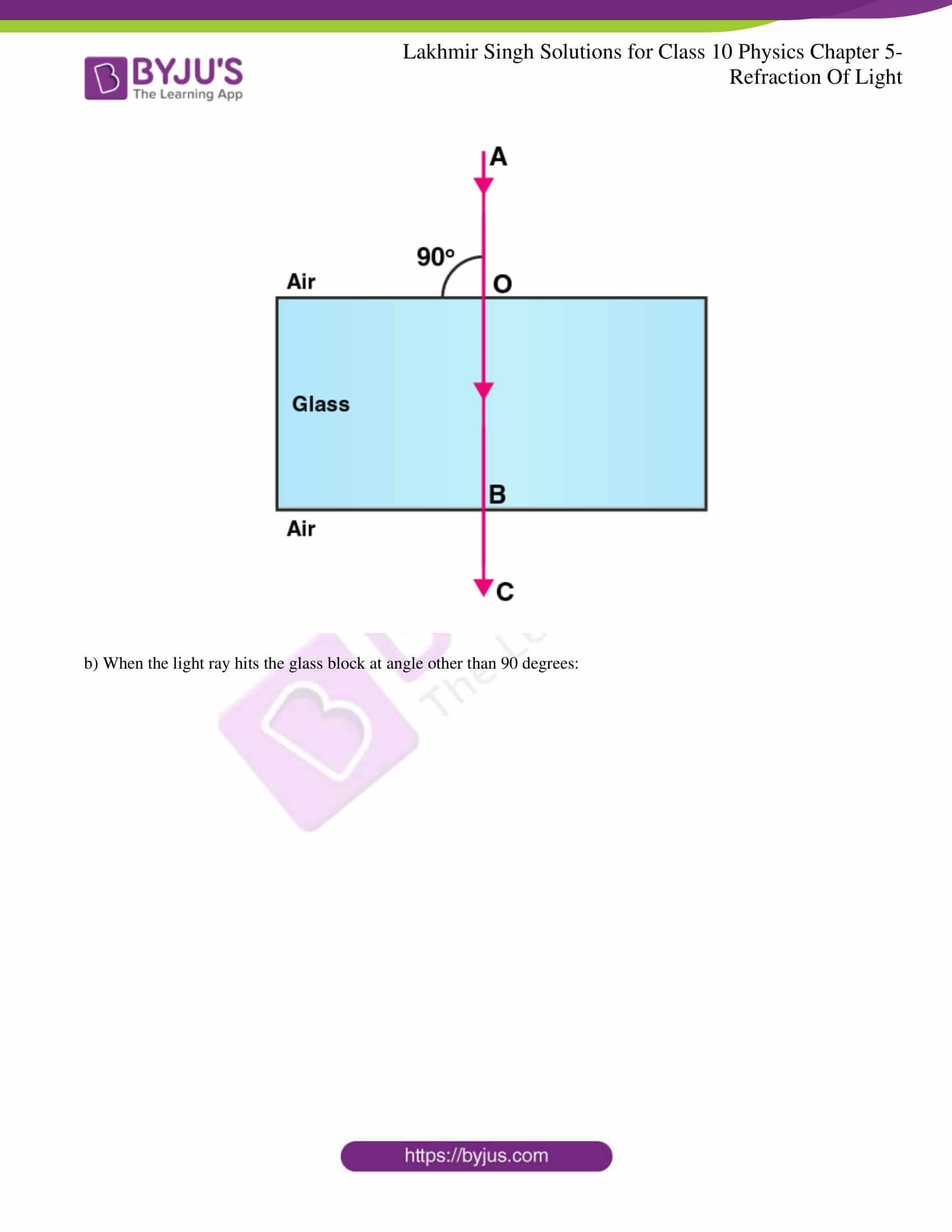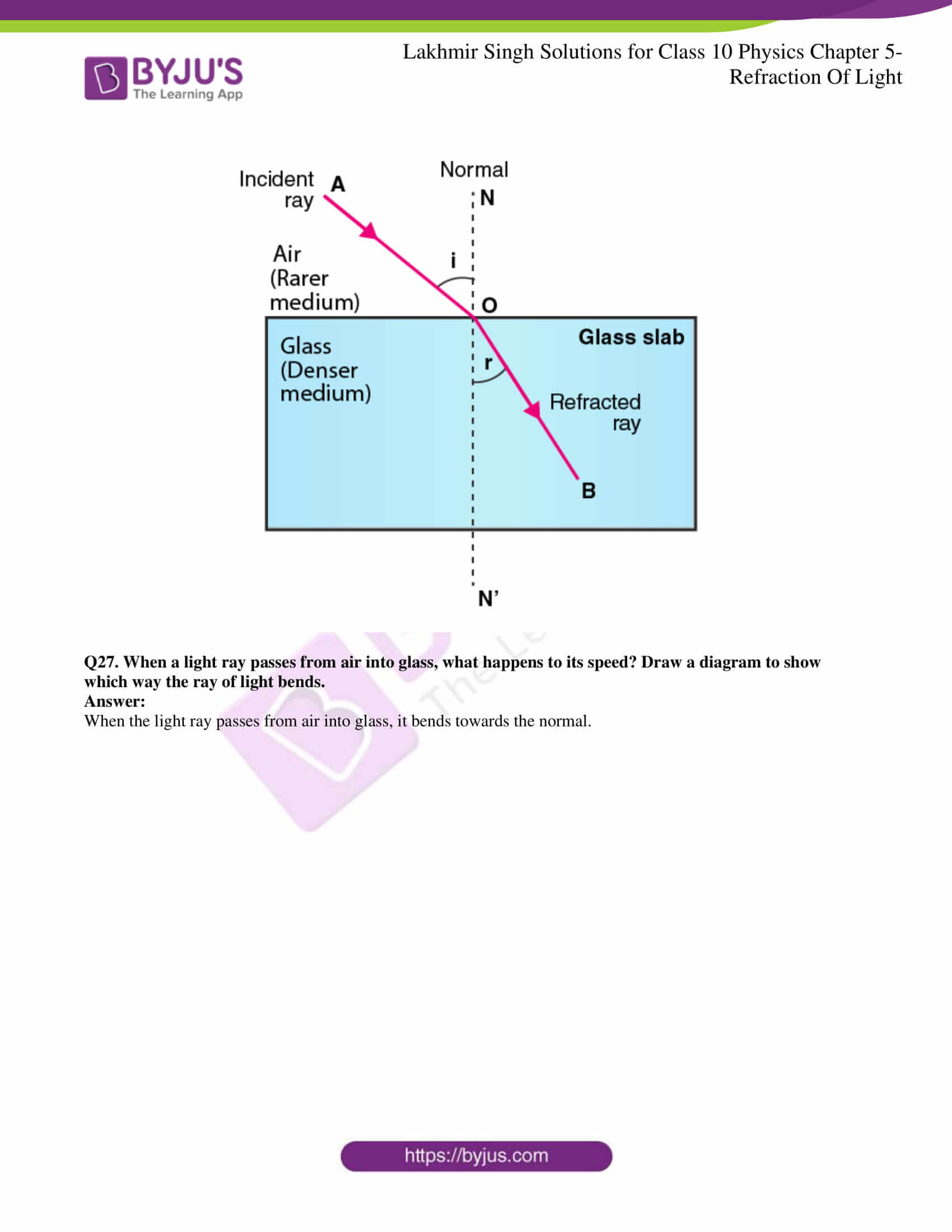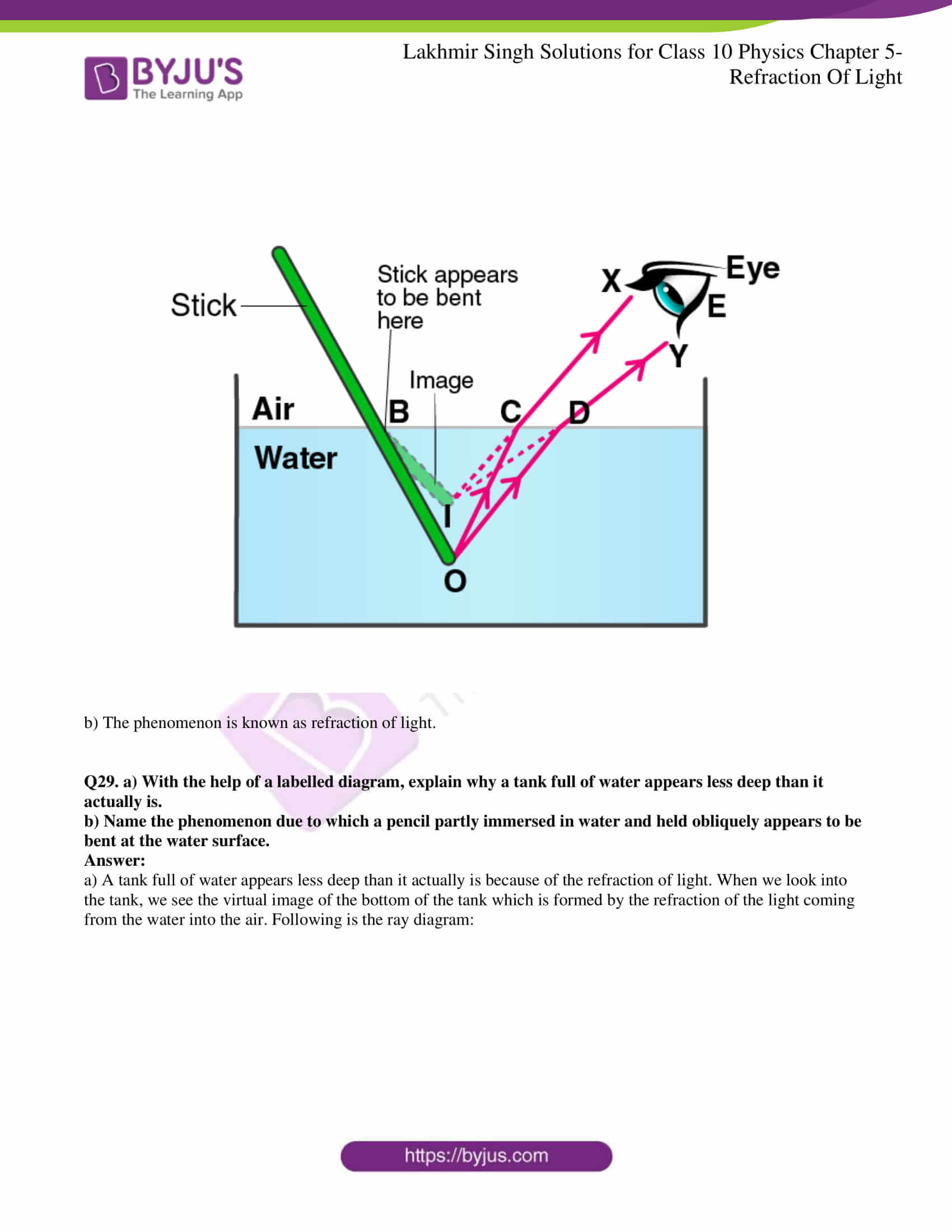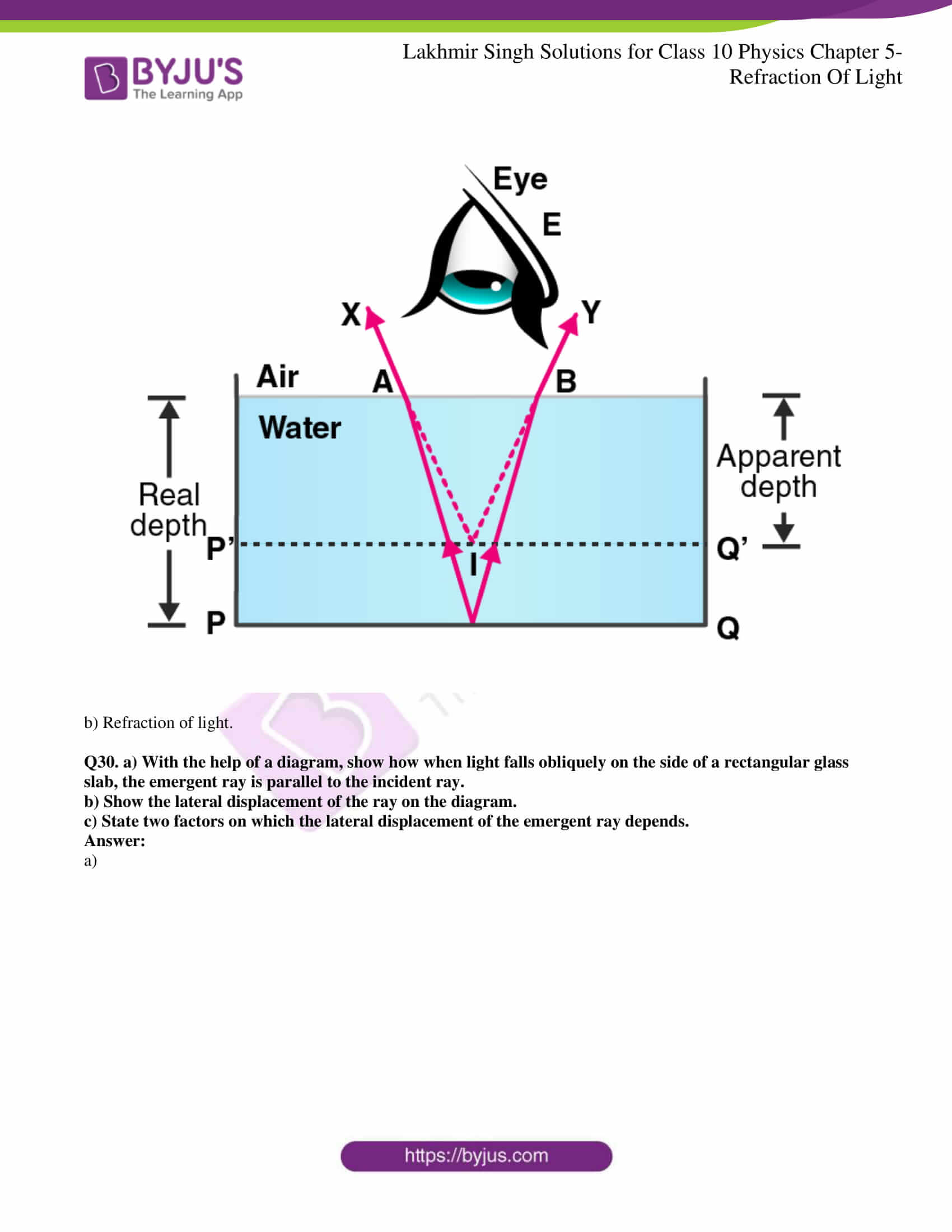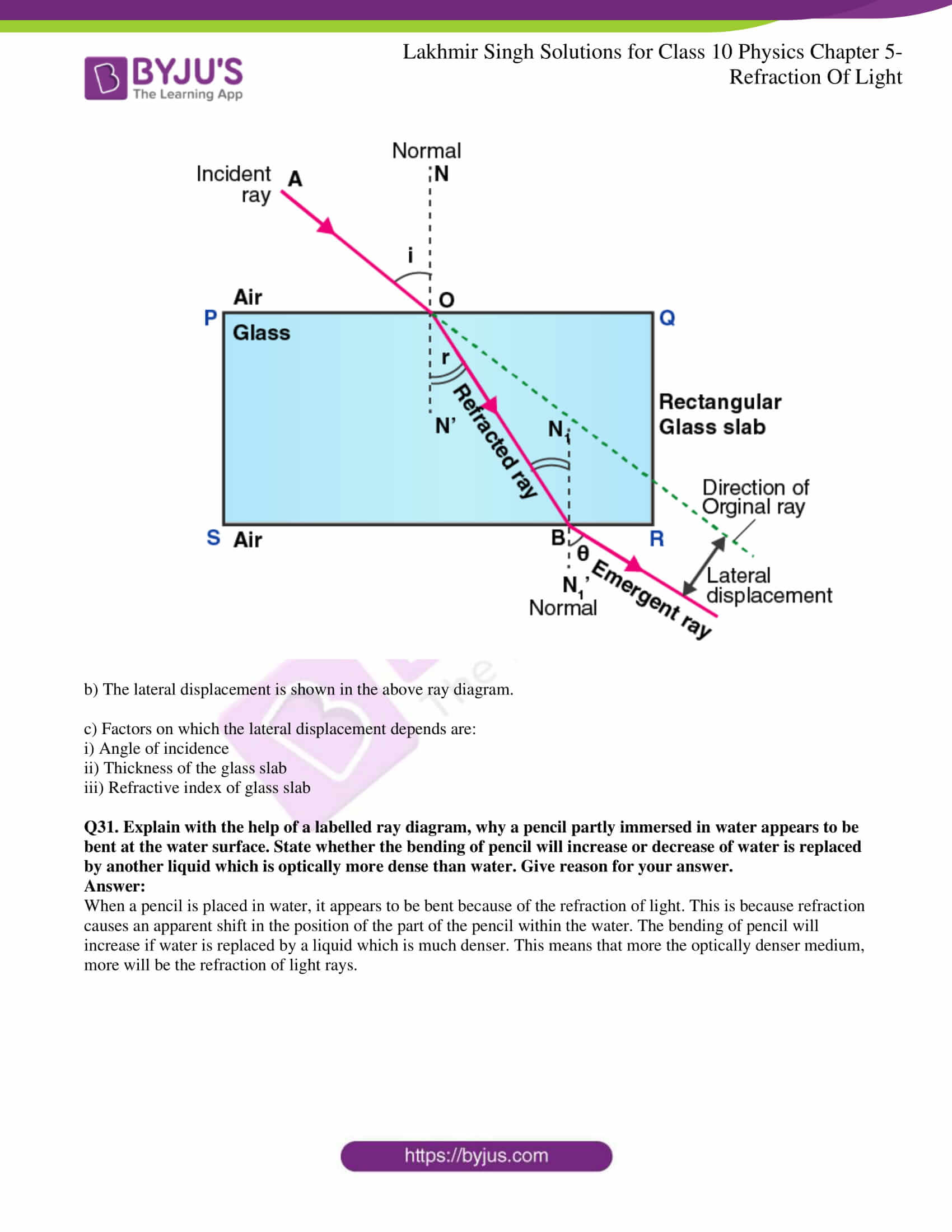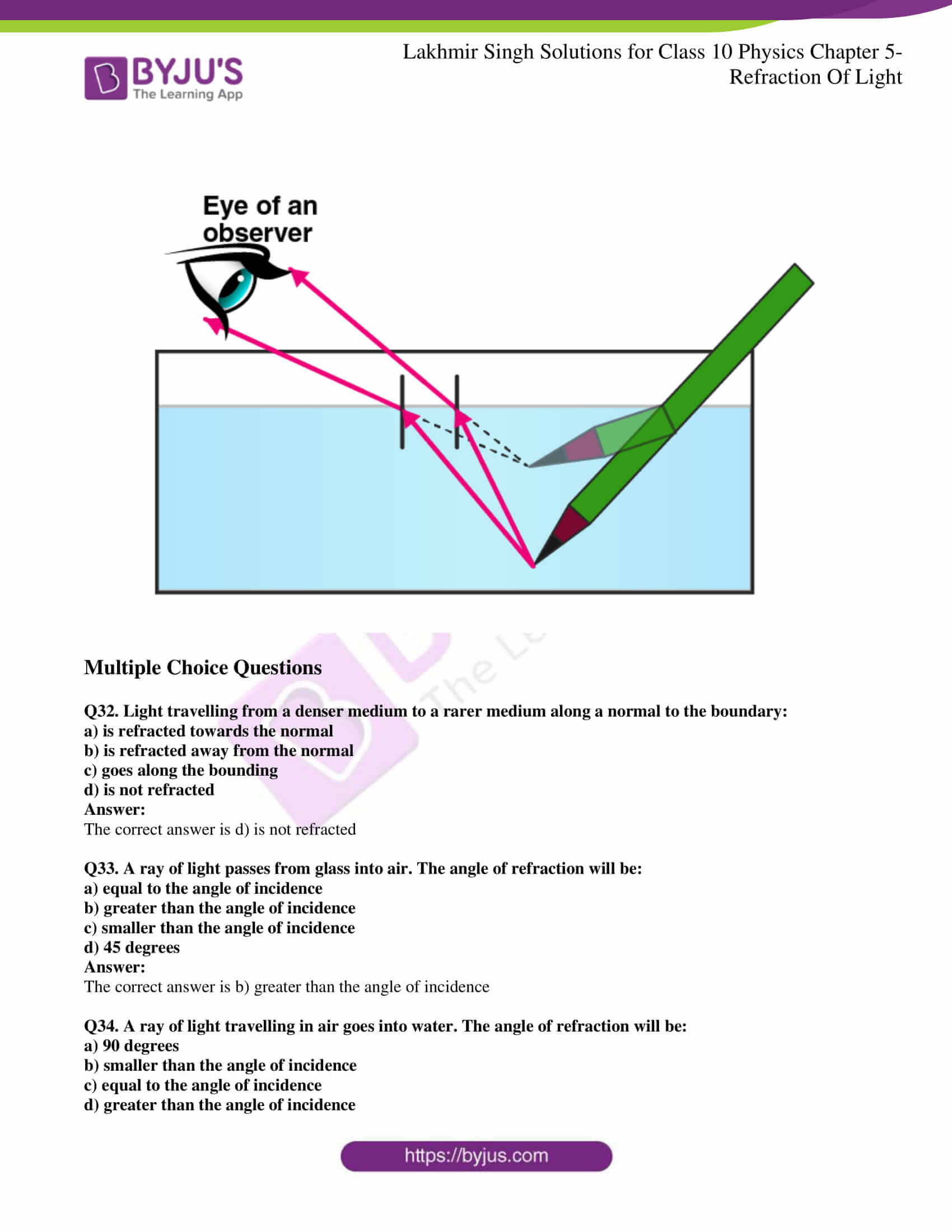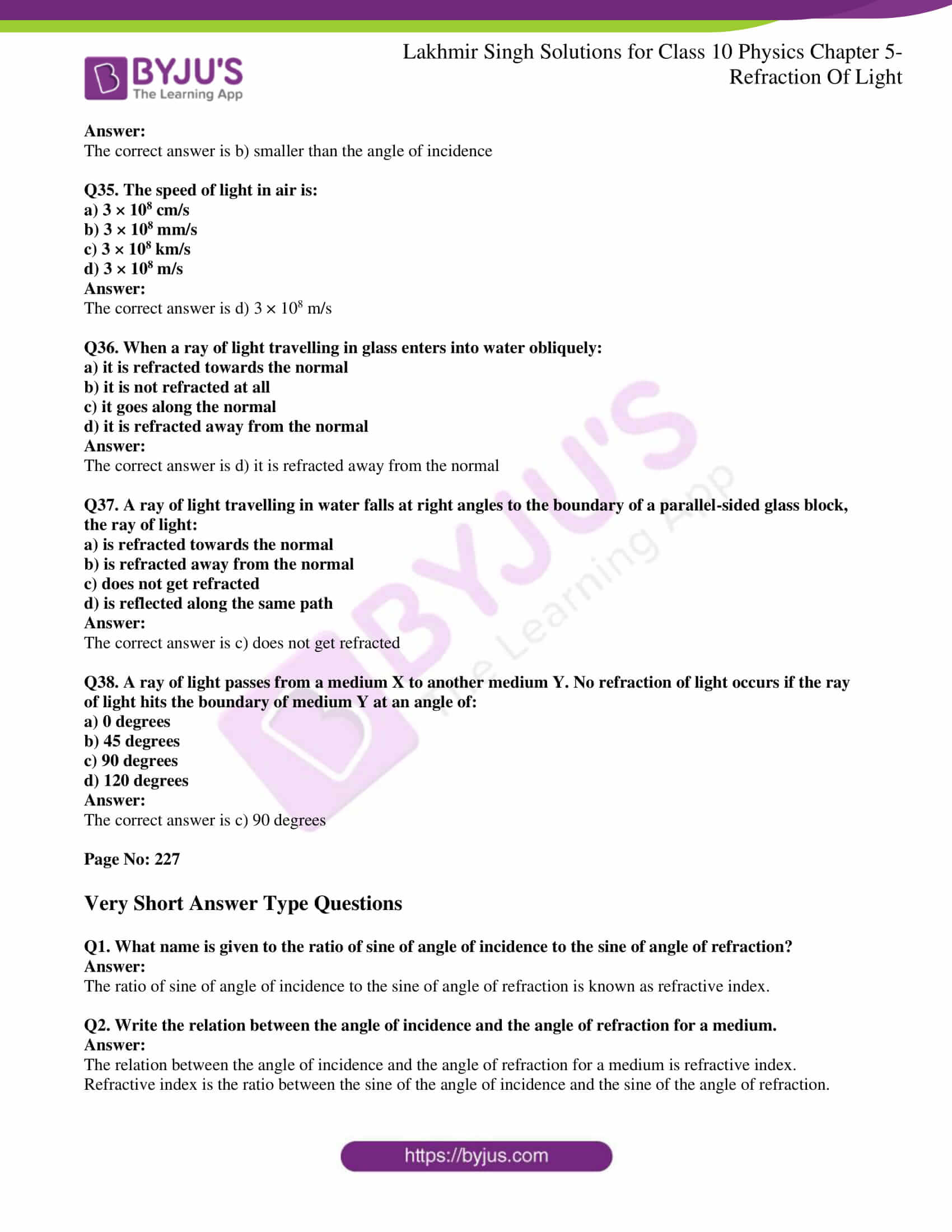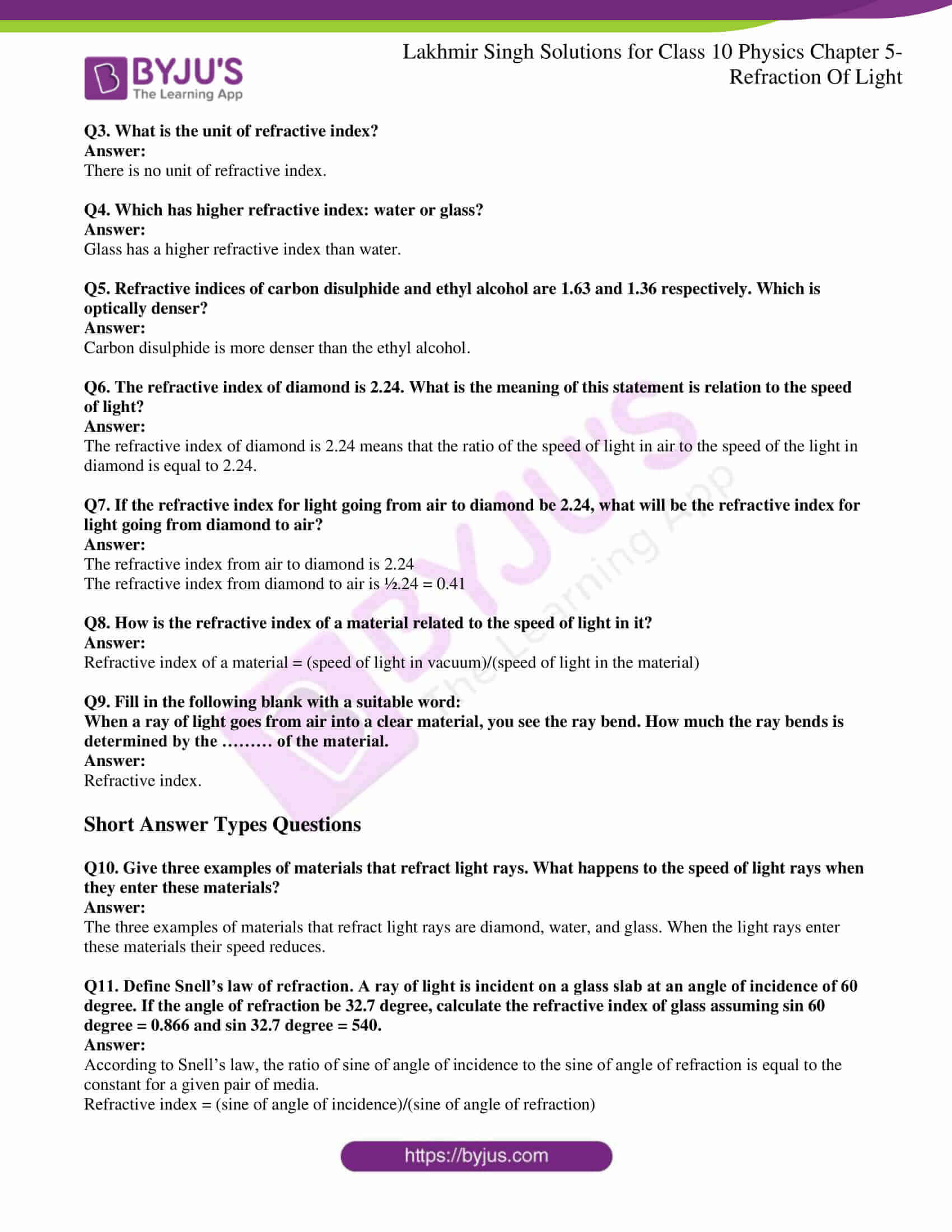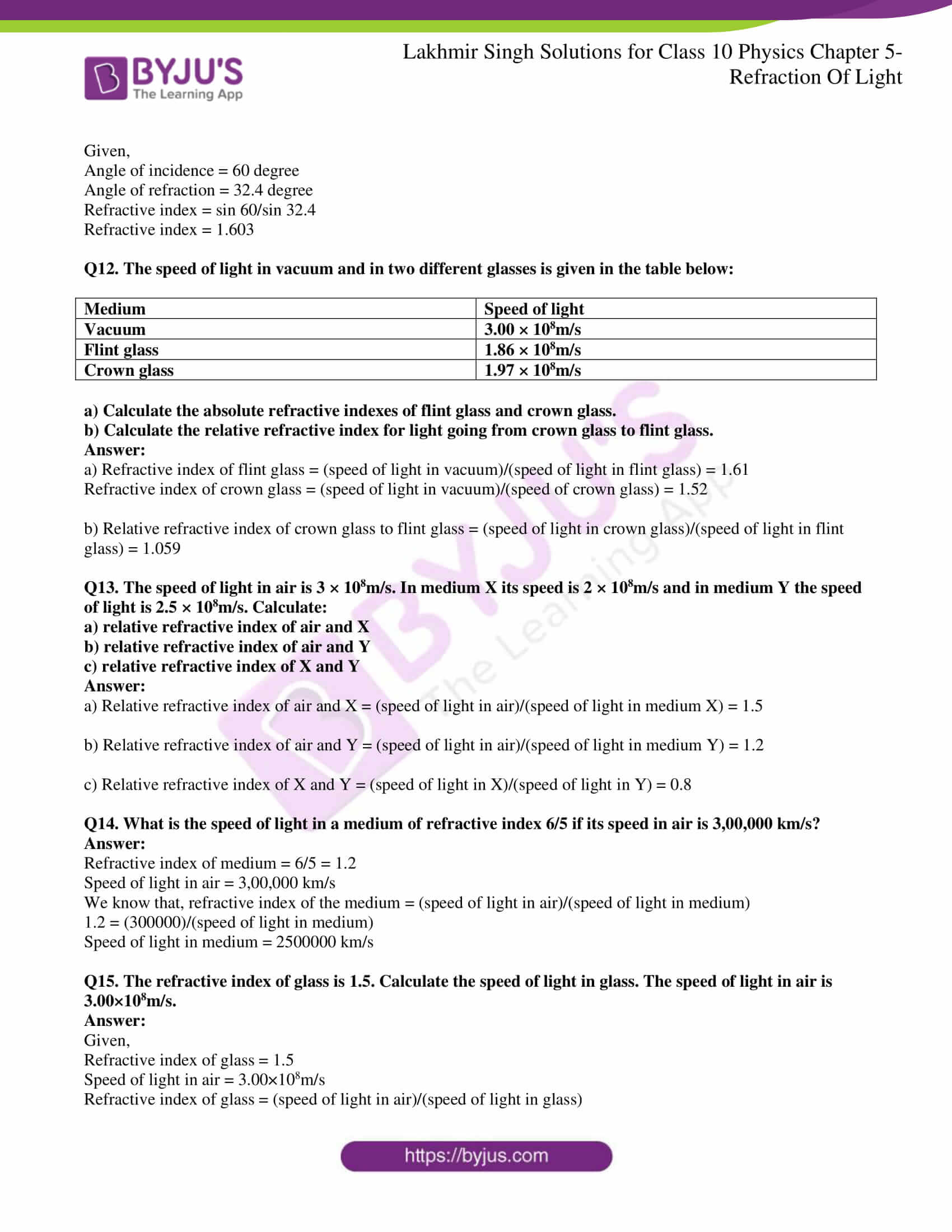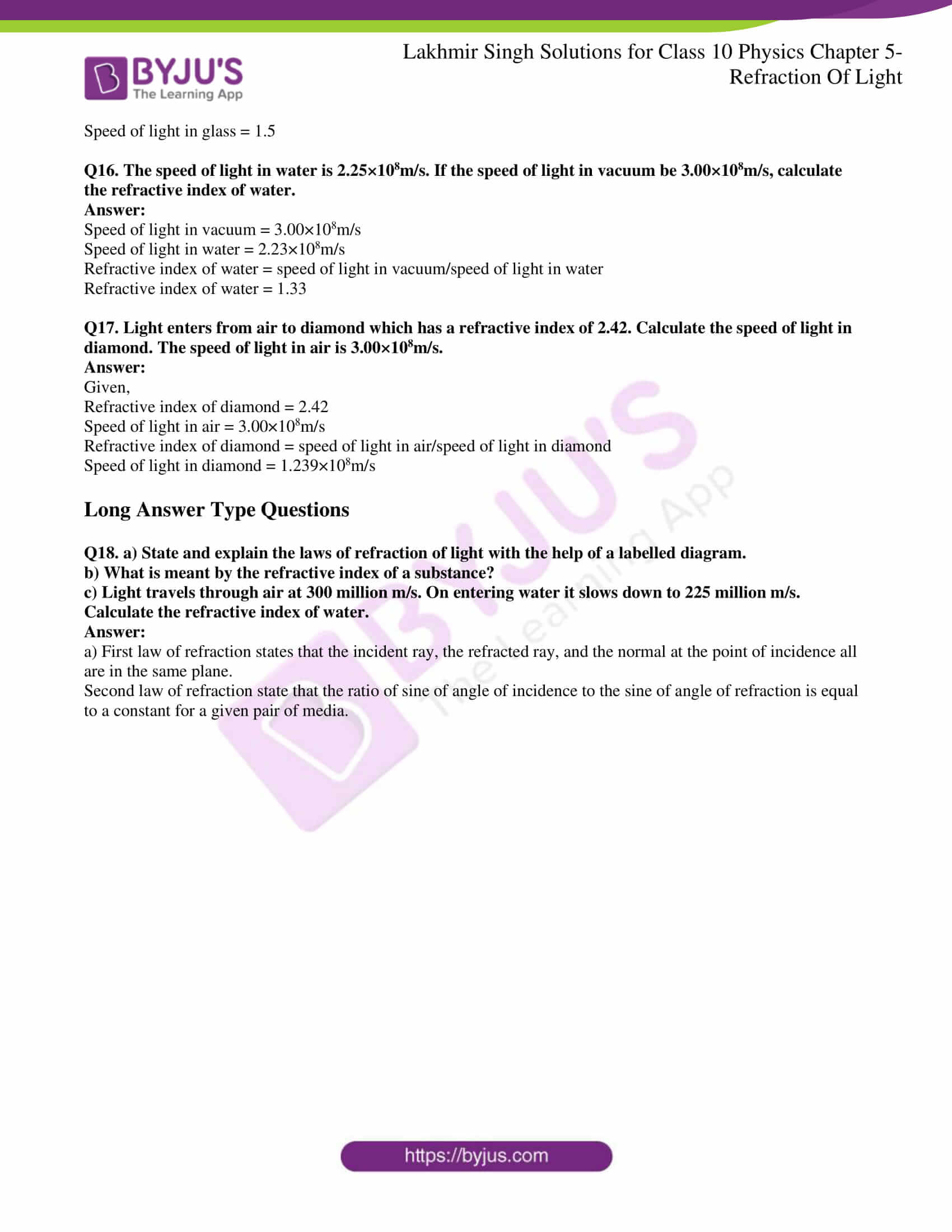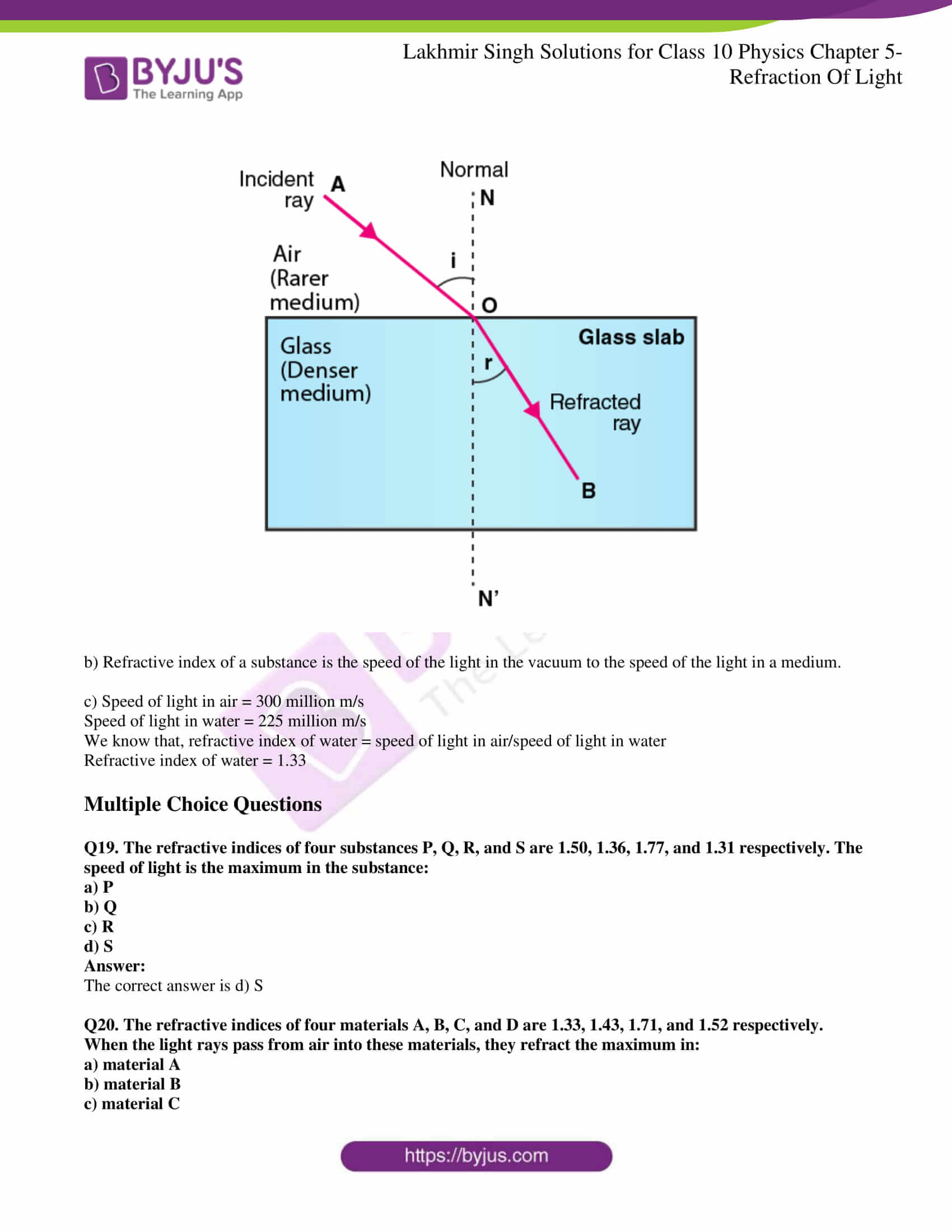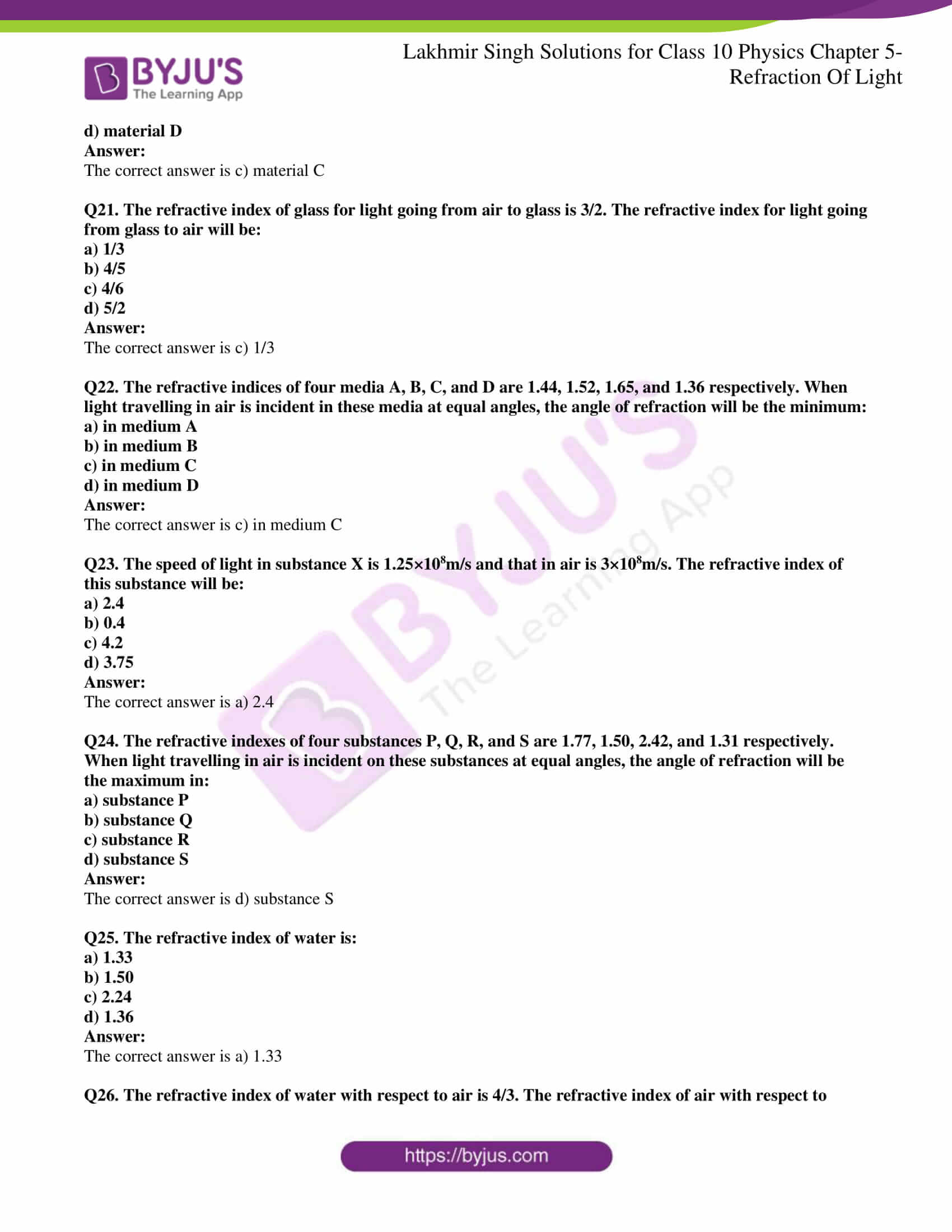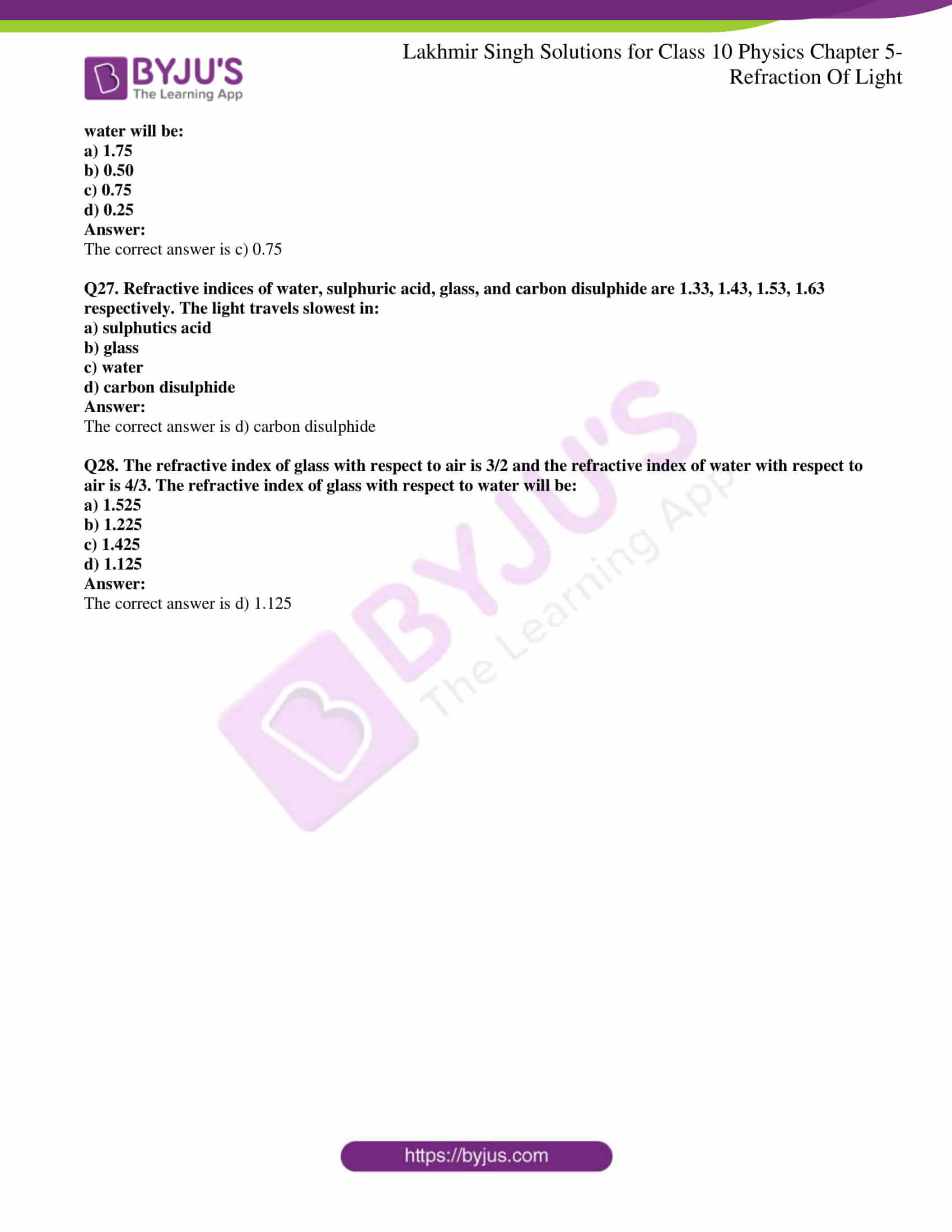Page No: 219

### Very Short Answer Type Questions

Q1. If a ray of light goes from a rarer medium to a denser medium, will it bend towards the normal or away from it?

The light ray will bend towards the normal.

Q2. If a ray of light goes from a denser medium to rare medium, will it bend towards the normal or away from the normal?

The light ray will bend away from the normal.

Q3. A beam of light travelling in a rectangular glass slab emerges into air. Draw a ray diagram indicating the change in its path.

Following is the ray diagram of a beam of light travelling in a rectangular glass slab emerging into air.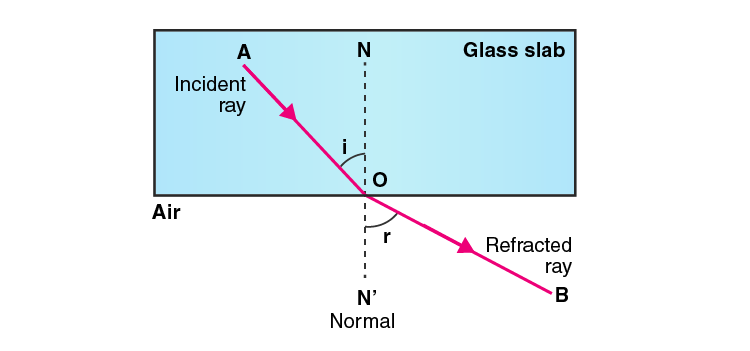Q4. A beam of light travelling in air is incident on water. Draw a ray diagram indicating the change in its path in water.

Following is the ray diagram of a beam of light travelling in air which is incident on water: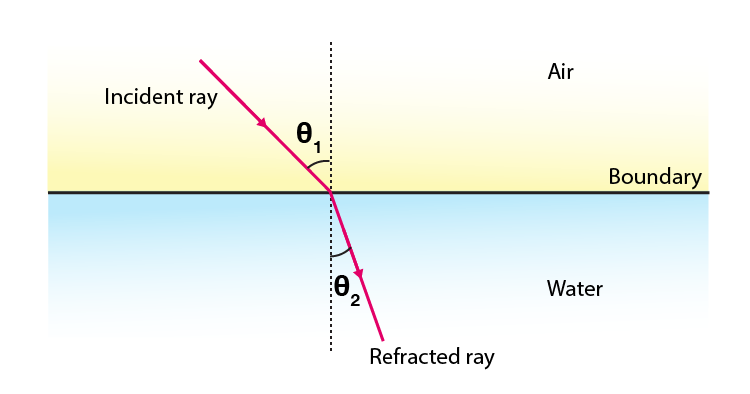Q5. A ray of light travelling in water emerges into air. Draw a ray diagram indicating the change in its path.

Following is the ray of light travelling in water emerging into air: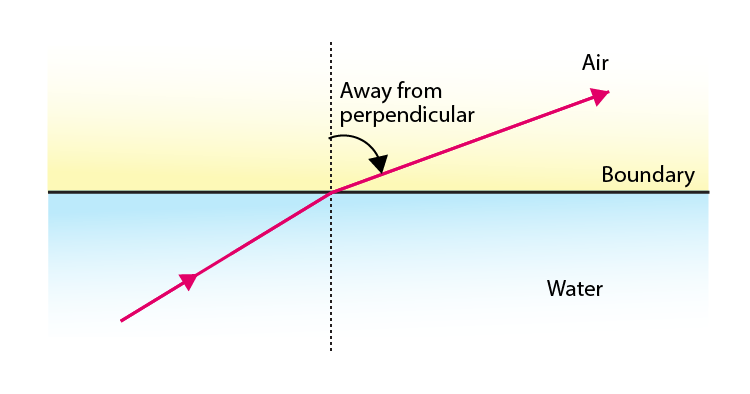Q6. A ray of light travelling in air is incident on a parallel-sided glass slab. Draw a ray diagram indicating the change in its path inn glass.

Following is the ray of light travelling in air which is incident on a parallel-sided glass slab: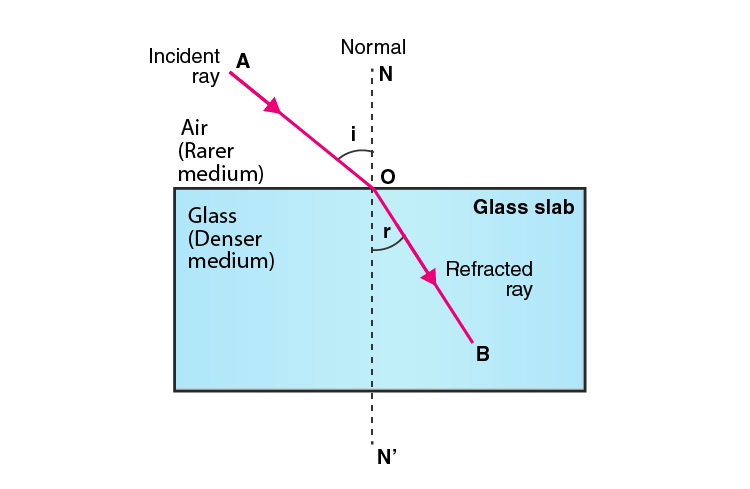Q7. A ray of light travelling in glass emerges into air. State whether it will bend towards the normal or away from the normal.

When the light ray is travelling in a glass emerging into air, the ray will bend away from the normal.

Q8. A ray of light travelling in air enters obliquely into water. Does the ray of light bend towards the normal or away from the normal? Why?

When the light ray is travelling in air entering into water obliquely, the light ray bends towards the normal because water as a medium has an optically denser medium than air.

Q9. A ray of light goes from water into air. Will it bend towards the normal or away from the normal?

When the light ray goes from water into air, the light ray will bend away from the normal.

Q10. State two effects caused by the refraction of light.

Following are the two effects caused by the refraction of light:

a) An object placed under water appears to be raised.

b) A pool water appears to be less deeper than it is in reality.

Q11. Name the phenomenon due to which a swimming pool appears less deep than it really is.

The phenomenon is known as refraction of light.

Q12. When a ray of light passes from air into glass, is the angle of refraction greater than or less than the angle of incidence?

When the ray of light passes from air into glass, the angle of refraction is less than the angle of incidence.

Q13. A ray of light passes from air into a block of glass. Does it bend towards the normal or away from it?

When a ray of light passes from air into a block of glass, the ray will bend towards the normal.

Q14. As light rays pass from water into glass, are they refracted towards the normal or away from the normal?

When the light rays pass from water into glass, they bend towards the normal.

Q15. In which material do you think light rays travel faster: glass or air?

The light rays travels faster in air when compared to the glass.

Q16. Which phenomenon of light makes the water to appear shallower than it really is?

Refraction of light is the phenomenon that makes the water to appear shallower than it really is.

Q17. State whether the following statement is true or false:

Refraction occurs because light slows down in denser materials.

The given statement is true.

Q18. Why does a ray of light bend when it travels from one medium to another?

A ray of light bends from one medium to another when it travels because there is a change in the speed of the light.

Q19. Fill in the following blanks with suitable words:

a) Light travelling along a normal is ……….

b) Light bends when it passes from water into air. We say that it is …………

a) Not

b) Refracted

Q20. What is meant by refraction of light? Draw a labelled ray diagram to show the refraction of light.

The change in direction of light when it travels from one medium to another is known as refraction of light.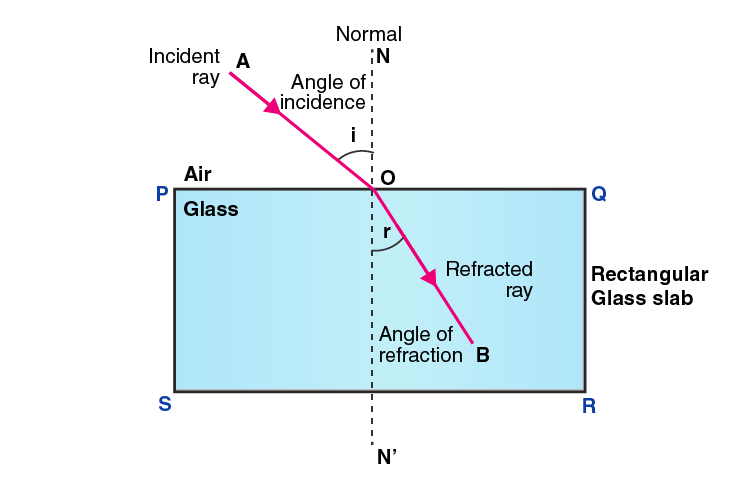Q21. A ray of light travelling in air is incident on a rectangular glass block and emerges out into the air from the opposite face. Draw a labelled ray diagram to show the complete path of this ray of light. Mark the two points where the refraction of light takes place. What can you say about the final direction of ray of light?

The final direction of the ray of light will be the same as the incident direction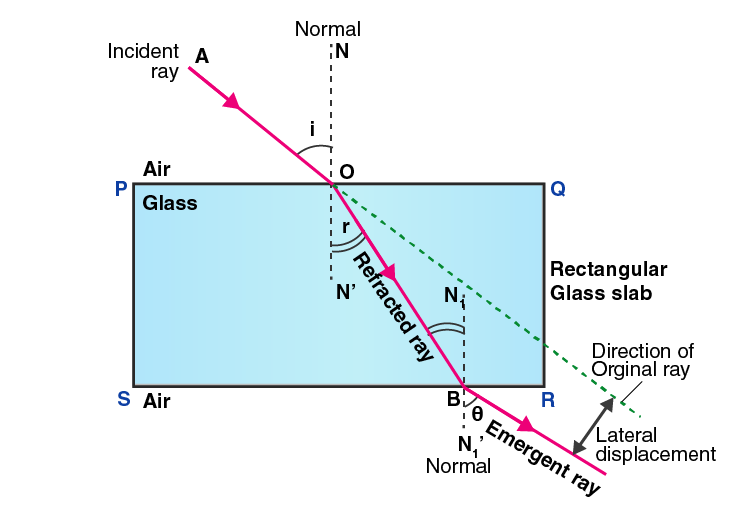Q22. Draw a labelled ray diagram to show how a ray of light is refracted when it passes:

a) from air into an optically denser medium

b) from an optically denser medium into air

a) Following is the ray diagram of a ray of light travelling from air into an optically denser medium:b) Following is the ray diagram of light travelling from an optically denser medium into air:Q23. The diagram given alongside shows a ray of light entering a rectangular block of glass.

a) Copy the diagram and draw the normal at the point of entry.

b) Draw the approximate path of the ray of light through the glass block and out of the other side.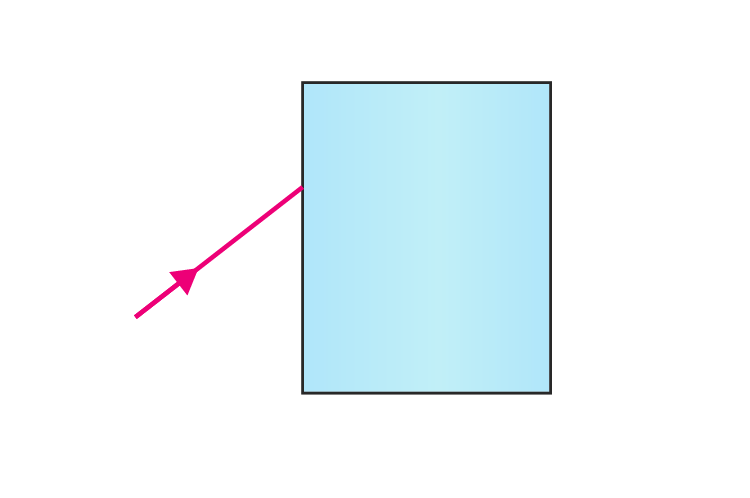a)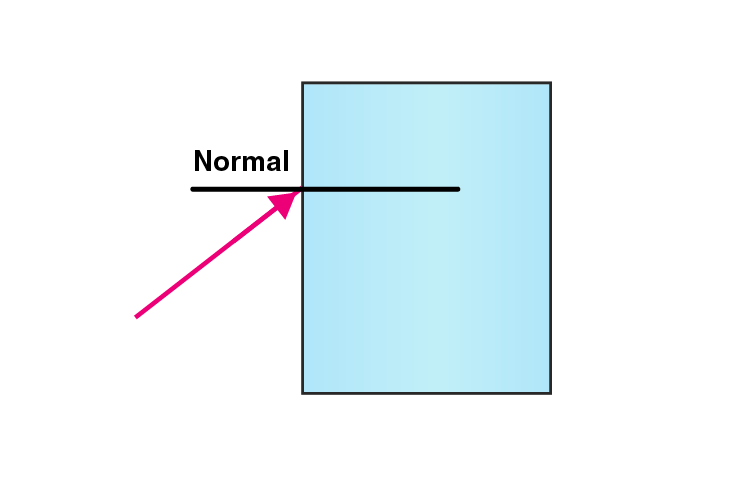b)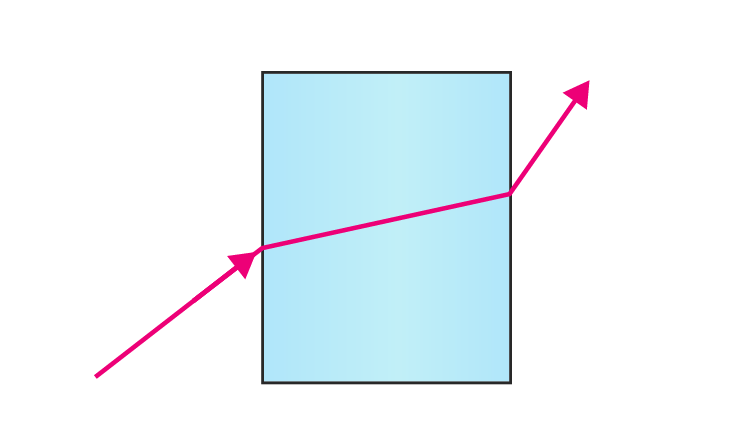Q24. What is meant by the angle of incidence and the angle of refraction for a ray of light? Draw a labelled ray diagram to show the angle of incidence and the angle of refraction for a refracted ray of light.

The angle of incidence is defined as the angle between the incident ray and the normal at the point of incidence.

The angle of refraction is defined as the angle between the refracted ray and the normal at the point of refraction.Q25. Light travels more quickly through water than through glass.

a) Which is optically denser: water or glass?

b) If a ray of light passes from glass into water, which way will it bend: towards the normal or away from the normal?

a) Glass is optically denser than water.

b) If a ray of light is passing from glass into water, it will bend away from the normal.

Q26. Draw a labelled ray diagram to show how a ray of light passes through a parallel sided glass block:

a) If it hits the glass block at 90 degrees that is perpendicular to the glass block

b) If it hits the glass block at an angle other than 90 degrees that is obliquely to the glass block

a) When the light ray hits the glass block at 90 degrees: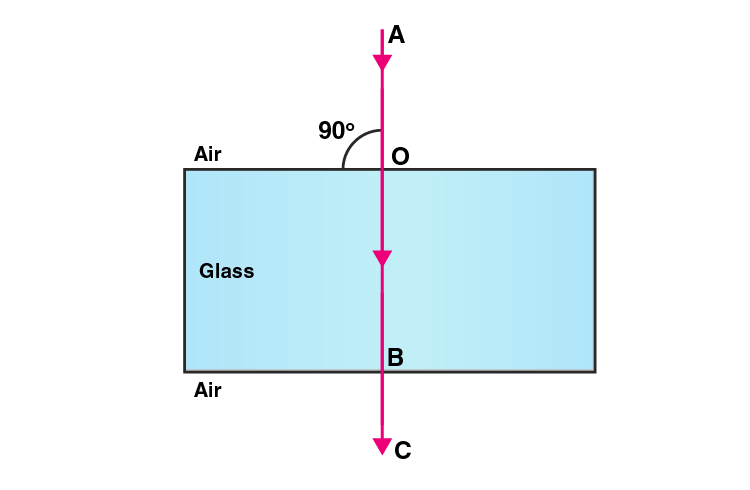b) When the light ray hits the glass block at angle other than 90 degrees:Q27. When a light ray passes from air into glass, what happens to its speed? Draw a diagram to show which way the ray of light bends.

When the light ray passes from air into glass, it bends towards the normal.Q28. a) Explain why a stick half immersed in water appears to be bent at the surface. Draw a labelled diagram to illustrate your answer.

b) A coin in a glass tumbler appears to rise as the glass tumbler is slowly filled with water. Name the phenomenon responsible for this effect.

a) The stick appears to be bent at the surface because of refraction of light.

Let OC be the ray coming from O which passes from water into air and gets refracted away from the normal. OD is the second ray that gets refracted along with DY. Therefore, the two refracted rays are CX and DY which appears to meet at point I. Therefore, I is the virtual image at the end of O. Following is the ray diagram: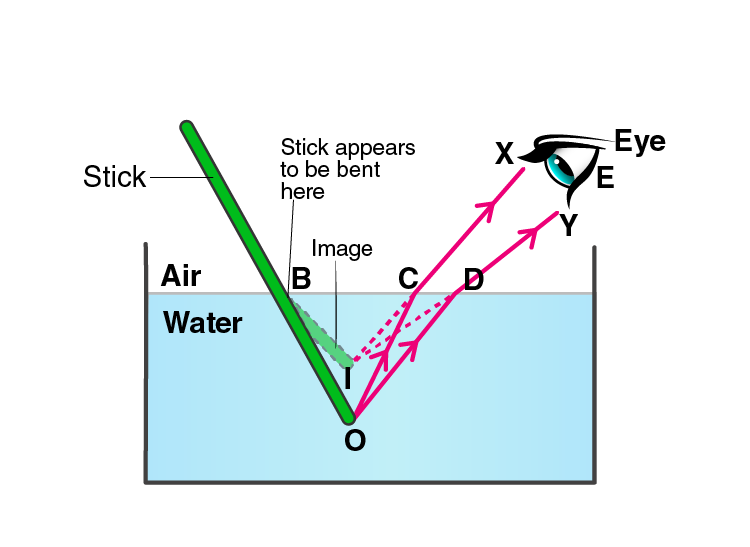b) The phenomenon is known as refraction of light.

Q29. a) With the help of a labelled diagram, explain why a tank full of water appears less deep than it actually is.

b) Name the phenomenon due to which a pencil partly immersed in water and held obliquely appears to be bent at the water surface.

a) A tank full of water appears less deep than it actually is because of the refraction of light. When we look into the tank, we see the virtual image of the bottom of the tank which is formed by the refraction of the light coming from the water into the air. Following is the ray diagram: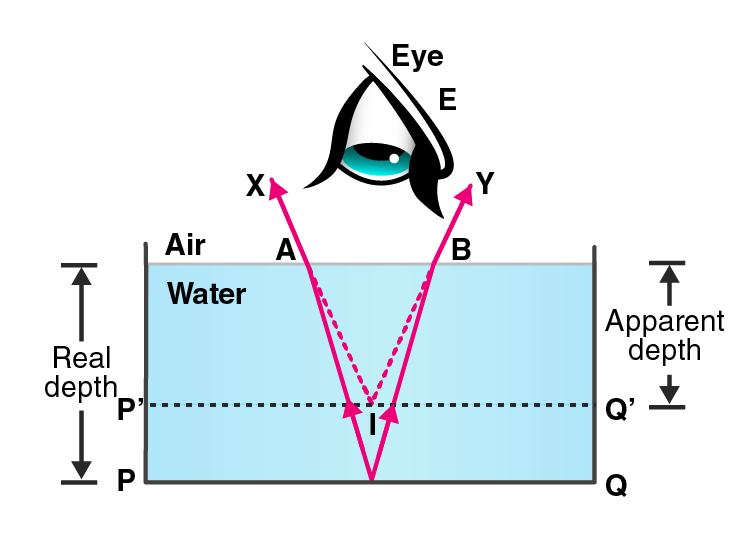b) Refraction of light.

Q30. a) With the help of a diagram, show how when light falls obliquely on the side of a rectangular glass slab, the emergent ray is parallel to the incident ray.

b) Show the lateral displacement of the ray on the diagram.

c) State two factors on which the lateral displacement of the emergent ray depends.

a)b) The lateral displacement is shown in the above ray diagram.

c) Factors on which the lateral displacement depends are:

i) Angle of incidence

ii) Thickness of the glass slab

iii) Refractive index of glass slab

Q31. Explain with the help of a labelled ray diagram, why a pencil partly immersed in water appears to be bent at the water surface. State whether the bending of pencil will increase or decrease of water is replaced by another liquid which is optically more dense than water. Give reason for your answer.

When a pencil is placed in water, it appears to be bent because of the refraction of light. This is because refraction causes an apparent shift in the position of the part of the pencil within the water. The bending of pencil will increase if water is replaced by a liquid which is much denser. This means that more the optically denser medium, more will be the refraction of light rays.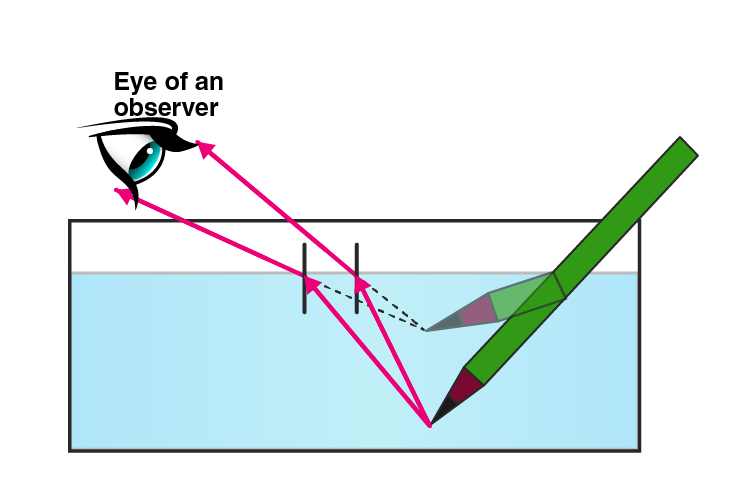### Multiple Choice Questions

Q32. Light travelling from a denser medium to a rarer medium along a normal to the boundary:

a) is refracted towards the normal

b) is refracted away from the normal

c) goes along the bounding

d) is not refracted

The correct answer is d) is not refracted

Q33. A ray of light passes from glass into air. The angle of refraction will be:

a) equal to the angle of incidence

b) greater than the angle of incidence

c) smaller than the angle of incidence

d) 45 degrees

The correct answer is b) greater than the angle of incidence

Q34. A ray of light travelling in air goes into water. The angle of refraction will be:

a) 90 degrees

b) smaller than the angle of incidence

c) equal to the angle of incidence

d) greater than the angle of incidence

The correct answer is b) smaller than the angle of incidence

Q35. The speed of light in air is:

a) 3 × 108 cm/s

b) 3 × 108 mm/s

c) 3 × 108 km/s

d) 3 × 108 m/s

The correct answer is d) 3 × 108 m/s

Q36. When a ray of light travelling in glass enters into water obliquely:

a) it is refracted towards the normal

b) it is not refracted at all

c) it goes along the normal

d) it is refracted away from the normal

The correct answer is d) it is refracted away from the normal

Q37. A ray of light travelling in water falls at right angles to the boundary of a parallel-sided glass block, the ray of light:

a) is refracted towards the normal

b) is refracted away from the normal

c) does not get refracted

d) is reflected along the same path

The correct answer is c) does not get refracted

Q38. A ray of light passes from a medium X to another medium Y. No refraction of light occurs if the ray of light hits the boundary of medium Y at an angle of:

a) 0 degrees

b) 45 degrees

c) 90 degrees

d) 120 degrees

The correct answer is c) 90 degrees

Page No: 227

### Very Short Answer Type Questions

Q1. What name is given to the ratio of sine of angle of incidence to the sine of angle of refraction?

The ratio of sine of angle of incidence to the sine of angle of refraction is known as refractive index.

Q2. Write the relation between the angle of incidence and the angle of refraction for a medium.

The relation between the angle of incidence and the angle of refraction for a medium is refractive index. Refractive index is the ratio between the sine of the angle of incidence and the sine of the angle of refraction.

Q3. What is the unit of refractive index?

There is no unit of refractive index.

Q4. Which has higher refractive index: water or glass?

Glass has a higher refractive index than water.

Q5. Refractive indices of carbon disulphide and ethyl alcohol are 1.63 and 1.36 respectively. Which is optically denser?

Carbon disulphide is more denser than the ethyl alcohol.

Q6. The refractive index of diamond is 2.24. What is the meaning of this statement is relation to the speed of light?

The refractive index of diamond is 2.24 means that the ratio of the speed of light in air to the speed of the light in diamond is equal to 2.24.

Q7. If the refractive index for light going from air to diamond be 2.24, what will be the refractive index for light going from diamond to air?

The refractive index from air to diamond is 2.24

The refractive index from diamond to air is ½.24 = 0.41

Q8. How is the refractive index of a material related to the speed of light in it?

Refractive index of a material = (speed of light in vacuum)/(speed of light in the material)

Q9. Fill in the following blank with a suitable word:

When a ray of light goes from air into a clear material, you see the ray bend. How much the ray bends is determined by the ……… of the material.

Refractive index.

Q10. Give three examples of materials that refract light rays. What happens to the speed of light rays when they enter these materials?

The three examples of materials that refract light rays are diamond, water, and glass. When the light rays enter these materials their speed reduces.

Q11. Define Snell’s law of refraction. A ray of light is incident on a glass slab at an angle of incidence of 60 degree. If the angle of refraction be 32.7 degree, calculate the refractive index of glass assuming sin 60 degree = 0.866 and sin 32.7 degree = 540.

According to Snell’s law, the ratio of sine of angle of incidence to the sine of angle of refraction is equal to the constant for a given pair of media.

Refractive index = (sine of angle of incidence)/(sine of angle of refraction)

Given,

Angle of incidence = 60 degree

Angle of refraction = 32.4 degree

Refractive index = sin 60/sin 32.4

Refractive index = 1.603

Q12. The speed of light in vacuum and in two different glasses is given in the table below:

 Medium Speed of light Vacuum 3.00 × 108m/s Flint glass 1.86 × 108m/s Crown glass 1.97 × 108m/s

a) Calculate the absolute refractive indexes of flint glass and crown glass.

b) Calculate the relative refractive index for light going from crown glass to flint glass.

a) Refractive index of flint glass = (speed of light in vacuum)/(speed of light in flint glass) = 1.61

Refractive index of crown glass = (speed of light in vacuum)/(speed of crown glass) = 1.52

b) Relative refractive index of crown glass to flint glass = (speed of light in crown glass)/(speed of light in flint glass) = 1.059

Q13. The speed of light in air is 3 × 108m/s. In medium X its speed is 2 × 108m/s and in medium Y the speed of light is 2.5 × 108m/s. Calculate:

a) relative refractive index of air and X

b) relative refractive index of air and Y

c) relative refractive index of X and Y

a) Relative refractive index of air and X = (speed of light in air)/(speed of light in medium X) = 1.5

b) Relative refractive index of air and Y = (speed of light in air)/(speed of light in medium Y) = 1.2

c) Relative refractive index of X and Y = (speed of light in X)/(speed of light in Y) = 0.8

Q14. What is the speed of light in a medium of refractive index 6/5 if its speed in air is 3,00,000 km/s?

Refractive index of medium = 6/5 = 1.2

Speed of light in air = 3,00,000 km/s

We know that, refractive index of the medium = (speed of light in air)/(speed of light in medium)

1.2 = (300000)/(speed of light in medium)

Speed of light in medium = 2500000 km/s

Q15. The refractive index of glass is 1.5. Calculate the speed of light in glass. The speed of light in air is 3.00×108m/s.

Given,

Refractive index of glass = 1.5

Speed of light in air = 3.00×108m/s

Refractive index of glass = (speed of light in air)/(speed of light in glass)

Speed of light in glass = 1.5

Q16. The speed of light in water is 2.25×108m/s. If the speed of light in vacuum be 3.00×108m/s, calculate the refractive index of water.

Speed of light in vacuum = 3.00×108m/s

Speed of light in water = 2.23×108m/s

Refractive index of water = speed of light in vacuum/speed of light in water

Refractive index of water = 1.33

Q17. Light enters from air to diamond which has a refractive index of 2.42. Calculate the speed of light in diamond. The speed of light in air is 3.00×108m/s.

Given,

Refractive index of diamond = 2.42

Speed of light in air = 3.00×108m/s

Refractive index of diamond = speed of light in air/speed of light in diamond

Speed of light in diamond = 1.239×108m/s

Q18. a) State and explain the laws of refraction of light with the help of a labelled diagram.

b) What is meant by the refractive index of a substance?

c) Light travels through air at 300 million m/s. On entering water it slows down to 225 million m/s. Calculate the refractive index of water.

a) First law of refraction states that the incident ray, the refracted ray, and the normal at the point of incidence all are in the same plane.

Second law of refraction state that the ratio of sine of angle of incidence to the sine of angle of refraction is equal to a constant for a given pair of media.b) Refractive index of a substance is the speed of the light in the vacuum to the speed of the light in a medium.

c) Speed of light in air = 300 million m/s

Speed of light in water = 225 million m/s

We know that, refractive index of water = speed of light in air/speed of light in water

Refractive index of water = 1.33

### Multiple Choice Questions

Q19. The refractive indices of four substances P, Q, R, and S are 1.50, 1.36, 1.77, and 1.31 respectively. The speed of light is the maximum in the substance:

a) P

b) Q

c) R

d) S

The correct answer is d) S

Q20. The refractive indices of four materials A, B, C, and D are 1.33, 1.43, 1.71, and 1.52 respectively. When the light rays pass from air into these materials, they refract the maximum in:

a) material A

b) material B

c) material C

d) material D

The correct answer is c) material C

Q21. The refractive index of glass for light going from air to glass is 3/2. The refractive index for light going from glass to air will be:

a) 1/3

b) 4/5

c) 4/6

d) 5/2

The correct answer is c) 1/3

Q22. The refractive indices of four media A, B, C, and D are 1.44, 1.52, 1.65, and 1.36 respectively. When light travelling in air is incident in these media at equal angles, the angle of refraction will be the minimum:

a) in medium A

b) in medium B

c) in medium C

d) in medium D

The correct answer is c) in medium C

Q23. The speed of light in substance X is 1.25×108m/s and that in air is 3×108m/s. The refractive index of this substance will be:

a) 2.4

b) 0.4

c) 4.2

d) 3.75

The correct answer is a) 2.4

Q24. The refractive indexes of four substances P, Q, R, and S are 1.77, 1.50, 2.42, and 1.31 respectively. When light travelling in air is incident on these substances at equal angles, the angle of refraction will be the maximum in:

a) substance P

b) substance Q

c) substance R

d) substance S

The correct answer is d) substance S

Q25. The refractive index of water is:

a) 1.33

b) 1.50

c) 2.24

d) 1.36

The correct answer is a) 1.33

Q26. The refractive index of water with respect to air is 4/3. The refractive index of air with respect to water will be:

a) 1.75

b) 0.50

c) 0.75

d) 0.25

The correct answer is c) 0.75

Q27. Refractive indices of water, sulphuric acid, glass, and carbon disulphide are 1.33, 1.43, 1.53, 1.63 respectively. The light travels slowest in:

a) sulphutics acid

b) glass

c) water

d) carbon disulphide

The correct answer is d) carbon disulphide

Q28. The refractive index of glass with respect to air is 3/2 and the refractive index of water with respect to air is 4/3. The refractive index of glass with respect to water will be:

a) 1.525

b) 1.225

c) 1.425

d) 1.125

The correct answer is d) 1.125

Also, visit Lakhmir Singh Solutions for Class 10 Physics to get complete solutions for all chapters.